# Theory of Thin-Film Field-Effect Transistors (TFT)

## (modeling TFT devices)

electrical characterization of TFTs

#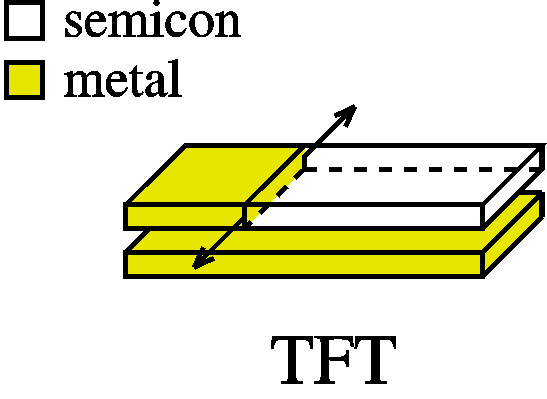These pages describe the theory behind thin-film transistors. Hope you enjoy it and, even more, hope it is useful to you. If you want to reference to this work (and help us get credit for it), please do so by citing the following papers for the complete work: Synthetic Metals 156, 1305 (2006)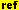, and Synthetic Metals 156, 1316 (2006). for the contacts: Organic Electronics 8, 300 (2007). for the effects of traps and the Meyer-Neldel Rule: Organic Electronics 7, 592 (2006). for the effects of water : Applied Physics Letters 88, 082101 (2006)for the metal transistor: Advanced Materials 20, 1 (2008)The complete work summarized in the book (see image on the right) Electrical Characterization of Organic Electronic Materials and Devices Peter Stallinga Wiley ISBN: 978-0-470-75009-4 Hardcover 316 pages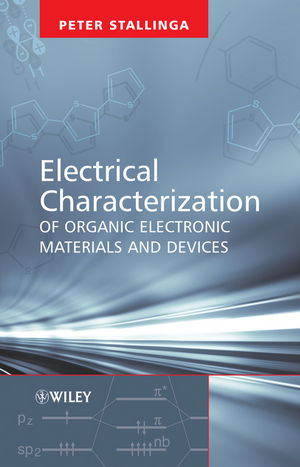Introductory remarks
Why a TFT is not a MOS-FET
The basic model
Linear region
Threshold voltage
Sub threshold current
Leakage current
Contact effects:
Contact resistance
Schottky barriers
Description of metal contacts in TFTs
Normally-on devices (coming soon)
Ambipolar devices
Metal-insulator-metal transistor
The effects of traps on the electrical characteristics of TFTs
IV curves (Poole-Frenkel)
Transfer curves (power law, Meyer-Neldel)
Current transients
Stressing
Peter Stallinga, May 2008.

## Introductory remarks

TFTs are best known for the consumer electronics components. Actually, 'TFT' is often confused with flat-panel display, like a CRT (cathode-ray tube) was confused with a old type of monitor or television. In fact, the TFT in these cases is the steering element of the display pixels, nothing more.
The advantages of TFTs over MOS-FETs is that their production is more flexible. With a common substrate, consisting of the gate, the insulator and the source and drain electrodes, rapidly many different types of TFTs based on different materials can be made. The device is then 'finalized' by depositing the active layer and this can be done from drop-casting, LB-film, spin-coating, up to vacuum deposition. All done with the same substrate. Such flexibility in production is especially interesting for research, of course.
The organic materials make full use of this flexibility, since they are easily solution processed and TFT fabrication is especially easy for organics. Some materials do not even need fancy environments (such as clean rooms, etc.) and can be dropcast from a pipet on top of the substrate to result in working and stable TFTs, exactly the kind of devices the organic area needs to aim at.

 Research spends a lot of effort on optimizing the performance of these devices. We have contributed to this by studying and modeling the TFT. What follows is a summary of our research. Probably the most pressing question is "What is the conduction mechanism?" (actually this question applies to all organic devices). Is it hopping conduction or band conduction? Unfortunately, it is not possible to decide between the two by simple electrical measurements. Often the 'mobility argument' is used as a referee. High mobility indicates band conduction and low mobility points at hopping conduction with 10-6-10-4 cm2/Vs being some sort of division line. However, we found out that mobilities in a very large range (10-8-1000 cm2/Vs) can be explained without change in conduction mechanism (Org. Electr. 7, 592 (2006)). In the following text, we use the nomenclature of band conduction (NV, NC, Eg, etc.), though we want to point out that it is just giving a name to the beasts. The only distinction that can be made is 'conductive states' and 'non-conductive states'. One might mean for instance valence band, but it can equally mean polarons. The other can be traps, but can equally mean polarons (!). It is also assumed that the mobility of conductive states is a fixed value (the 'intrinsic mobility') and that non-conductive states have zero mobility. Reality is probably more complicated. We justify these simplifications by the need to come up with a useful intuitive model that can easily be understood by a large community. However, in spite of this, the model that emerges is remarkably good for explaining actual data. On the other hand, if you came here for finding an answer to the question of the conduction model, you came to the wrong place.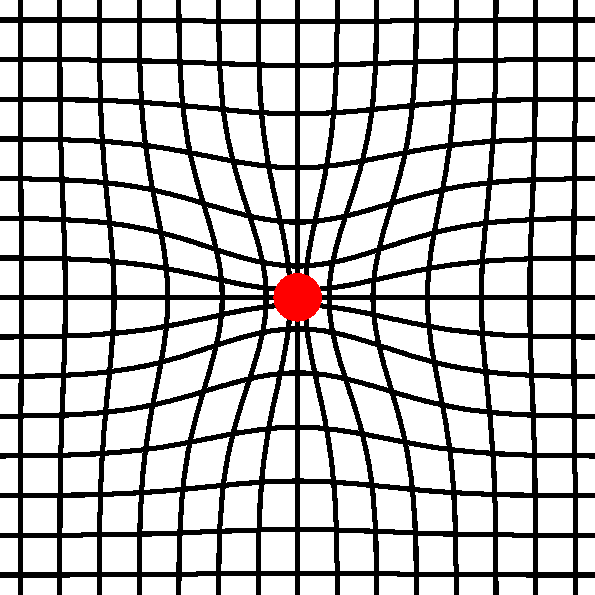Polaron; A charge inside it's self-created lattice distortion. Is it a conductive or non-conductive state?

## Why a TFT is not a MOS-FET

A MOS-FET is described in another section of these theory pages (MOS-FET)
• A MOS-FET works in inversion, meaning that the charge in the channel is of opposite sign compared to the bulk of the material. On the other hand, TFTs, work in accumulation (most organic semiconductors are p-type and most organic TFTs have hole-channels).
• In fact, even in a thick film transistor, when it is working in accumulation, all induced charge is at the interface and is free charge, simply because there are no electronic states to store immobile charge of the correct sign. Take as an example a p-type semiconductor; doped with acceptors NA. These acceptors can be neutral, or can accept an electron and become negatively charged. Now, when a bas is applied at the gate and an accumulation channel is created at the semiconductor side of the insulator, by definition, this channel has positive charge (holes) and the gate-bias is negative. Positive charge in the semiconductor can be only holes, because the acceptors can at best be neutral. Neutral charge does not contribute to any band bending (d2V/dx2 = r(x)) and does not add anything to the working of the device. The only charge induced in the semiconductor is therefore holes. But, since holes are free to move, they will migrate to the interface. This will continue until they hit the energetic barrier at the interface (the insulator should have a larger band gap than the semiconductor to prevent charge from leaking back to the metal) or when all the free charge states are full, when the hole density is equal to valence band density-of-states. This latter is not easy to achieve. A typical DOS of a monatomic layer of semiconducting material is large enough to receive all holes for all reasonable voltages.

These two discrepancies mentioned warrant a new model specially designed for TFTs (and MOS-FETs in accumulation). We developed such a model which we baptized "The Algarve Model for the TFT". The ideas are presented here. For the full description, please read our papers (Synthetic Metals 156, p.1305and p.1316).
The model is a strong simplification of reality. Reality is more complicated. However, it describes the reality very well and any model should always find an optimum balance between simplicity and descriptive power. I set out this journey not to make as good a description of devices as possible (good textbooks exist, for example Sze), but to come up with one that is as easy to understand by a large community. I tried to find the minimum requirements for a model to explain our (quite complicated) data measured in our lab. As such, the work was inspired by my great idols, William of Ockham (in the 14th century probably the first modern scientist when describing his Ockham's Razor) and Saint-Exupéry, both stating that models to describe nature should be as simple as possible. William of Ockham wrote it as (Wikipedia) "entia non sunt multiplicanda praeter necessitatem / entities should not be multiplied beyond necessity."; freely translated: "the explanation of any phenomenon should make as few assumptions as possible, eliminating those that make no difference in the observable predictions of the explanatory hypothesis or theory", thereby advocating using a 'razor' to cut away all the non-essential parts of the theory. Saint-Exupéry said more-or-less the same when he stated that "An engineer should be happy not when there is nothing left to add, but when there is nothing left to take out".
Thus, the best models are those that are simple while still maintaining an adequate description of reality. As an example, we assume all charge to be directly at the interface between the insulator and the semiconductor. In reality, the charge induced by the gate can be several layers distant from the interface (see the works of Horowitz, for example J. Mater. Res. 19, 1946 (2004)). These effects can be absorbed by the insulator capacitance Cox, which is then slightly smaller than the one calculated on basis of the insulator thickness and can even depend on the bias. However, these are minor perturbations and should be looked at only when the rest of the characterization of the device measured is under control. One should not get bogged down in insignificant details even if they are correct.
On the other hand, the simplicity of the model can directly show us some strong conclusions such as the impossibility to make a Schottky barrier at the contacts, as will be shown here, and a correct modeling of possible contact resistance, where these two are often used in literature to write off any undesired and not well understood behavior of the devices. Moreover, all sorts of gate-bias- and temperature dependent field-effect mobilities and distortions in IV curves are very easily found. Summarizing, while the modeling is simple, it is able to draw some very powerful conclusions.
I hope that the model can rapidly identify why your device is behaving in a particular peculiar way and that it may lead the way to removing the unwanted effects and paving the way for better devices. "If you don't know what is the problem you cannot find a solution".Directions: x: from source to drain y from oxide to surface of film z: along an electrode W: electrode width L: electrode distance dox: oxide thickness

## The basic model

The single postulate in the model is to treat the device as a simple parallel plate capacitor. All charge on both sides of the insulator is immediately at the interface, without band bendings or other ways to distribute the charge in another way. As such, the charge at any place in the device is directly proportional to the voltage drop at the insulator, like in a classical condensator (C = Q/V). In this simple starting model we will assume that all charge is composed of free holes only:

 r(x) = q p(x) = Cox [V(x)-Vg]                (1)

with r(x) the charge density at position x along the channel, Cox the oxide capacitance density (= eox/dox), V(x) the local potential in the channel and Vg the uniform potential at the gate. Note that the density of charge is per square meter, thus effectively treating the active layer as two-dimensional. At any point in the channel, the current Ix is then given by the product of the local free charge density, the local electrical field (Ex = -dV(x)/dx), the charge-carrier mobility m multiplied by the device width W. For instance, if the channel has only free holes, this equation would be:

 Ix(x) = -q p(x) m W dV(x)/dx                  (2)

For charge composed of only free-holes, r(x) = q p(x), as above, the differential equation can easily be solved when appropriate boundary conditions are used, namely V(0) =0, V(L) = Vds and Ix(x) = Ids for all x (indicating there are no current sources in the channel):

 Ids    = m Cox [VgVds - Vds2/2] W/L

which is equal to the behavior of a MOS-FET, explaining the persistence of the application of the MOS-FET model to TFT devices; that simple seems to be adequate. Further on we will show examples where the output curves deviate from simple MOS-FET behavior. We will show how the effective,as-measured mobility can be different from m and how it can depend on the bias (Vg and Vds) and the temperature (T).
The above equation is valid for drain-source biases up to Vg. After that point saturation starts. The same reasoning can be applied to TFTs as is common for MOS-FETs. When at the drain the potential is equal to the gate bias, no voltage drop exists there and no free charge (Eq. 1) either and the current is zero.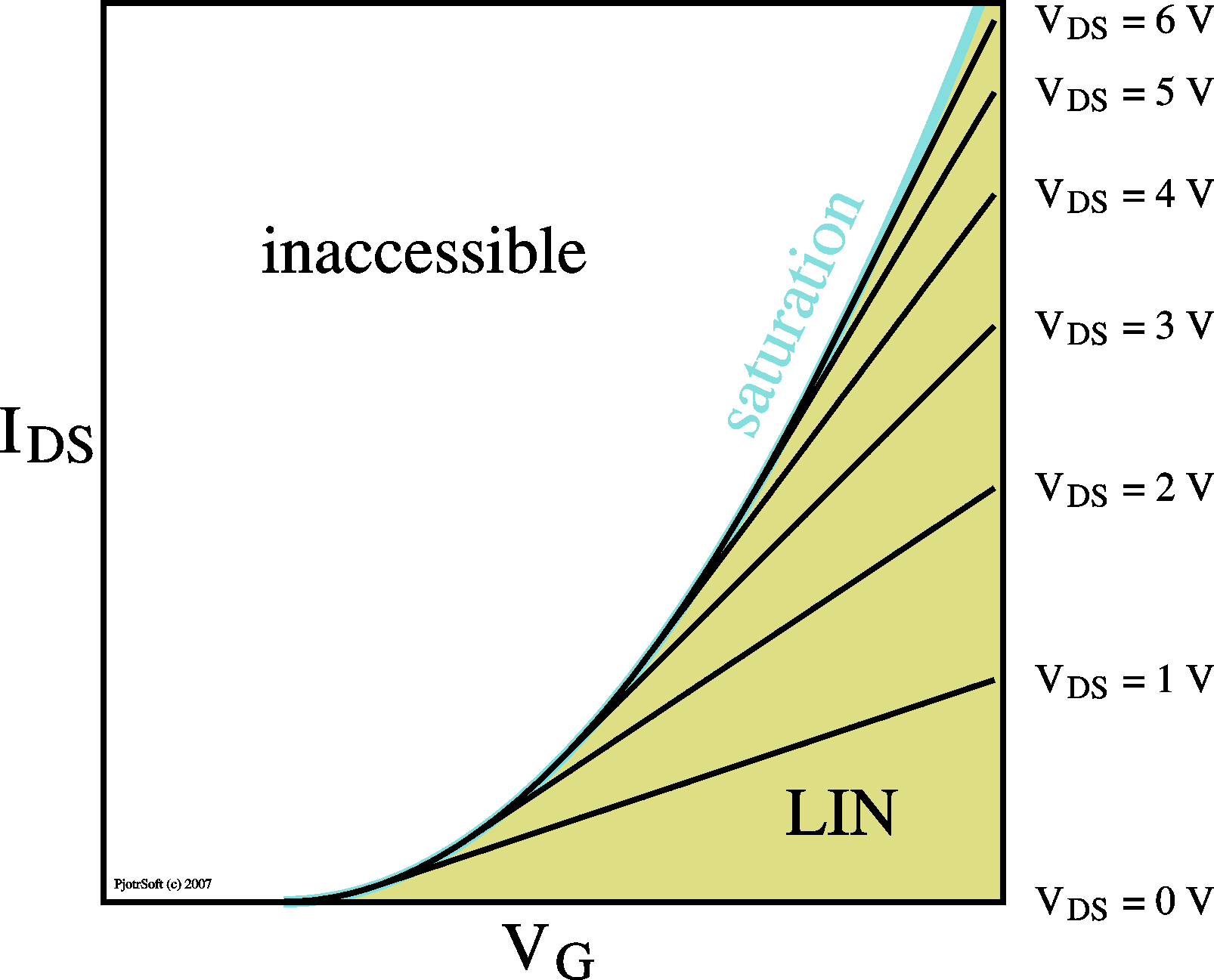Examples of IV curves (Ids vs. Vds; left) for different gate voltages and transfer curves (Ids vs. Vg; right) for different drain-source voltages.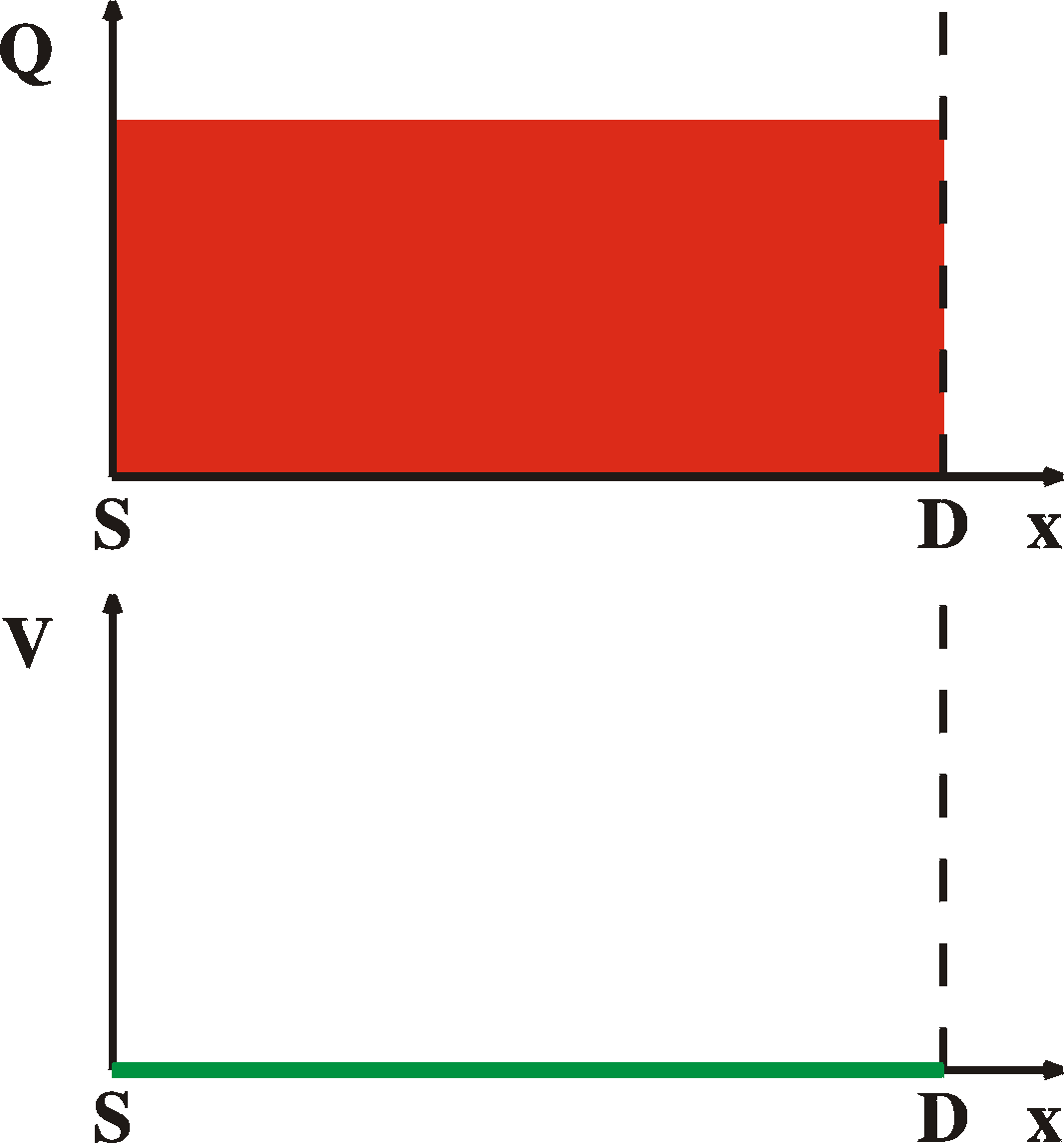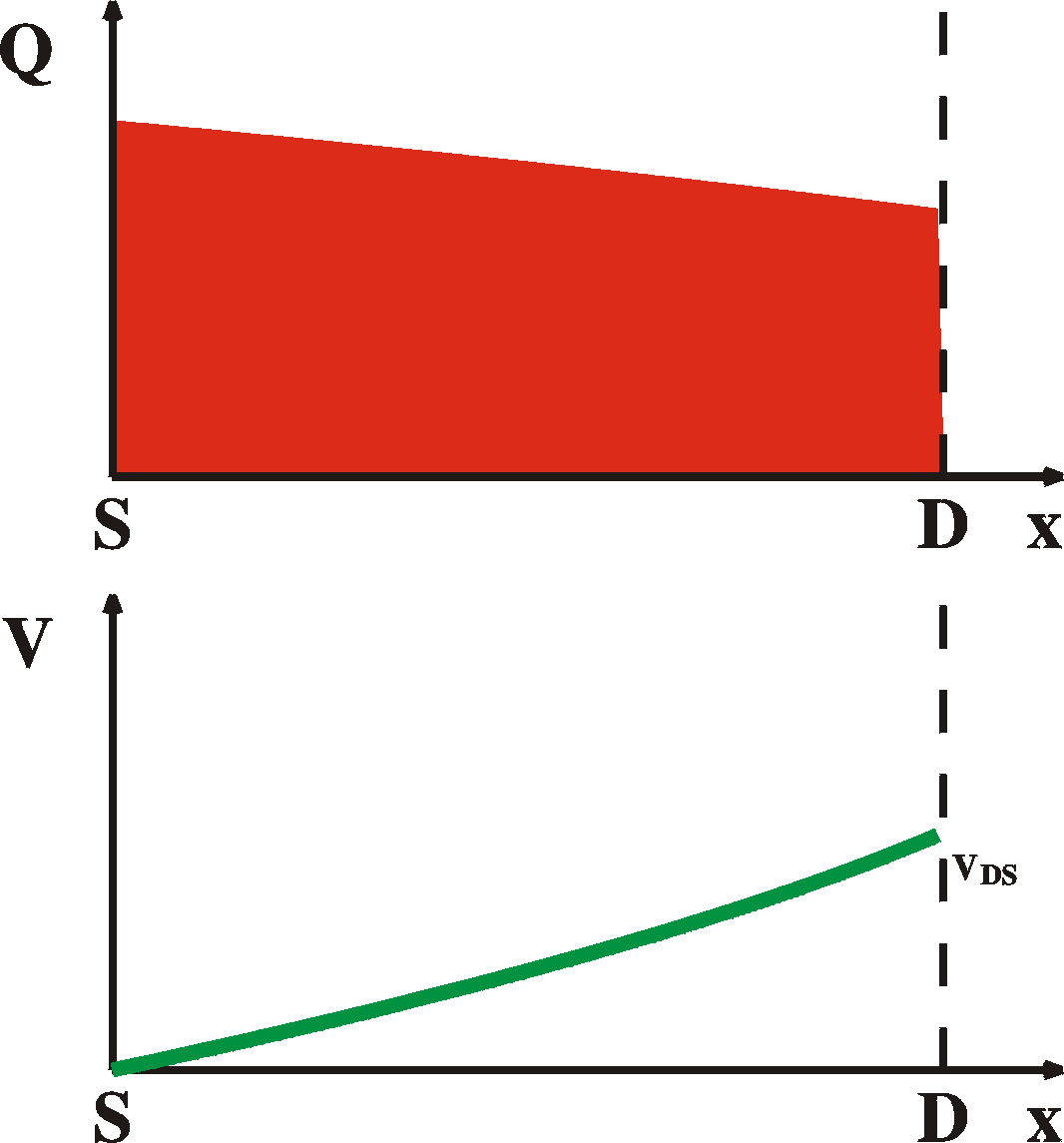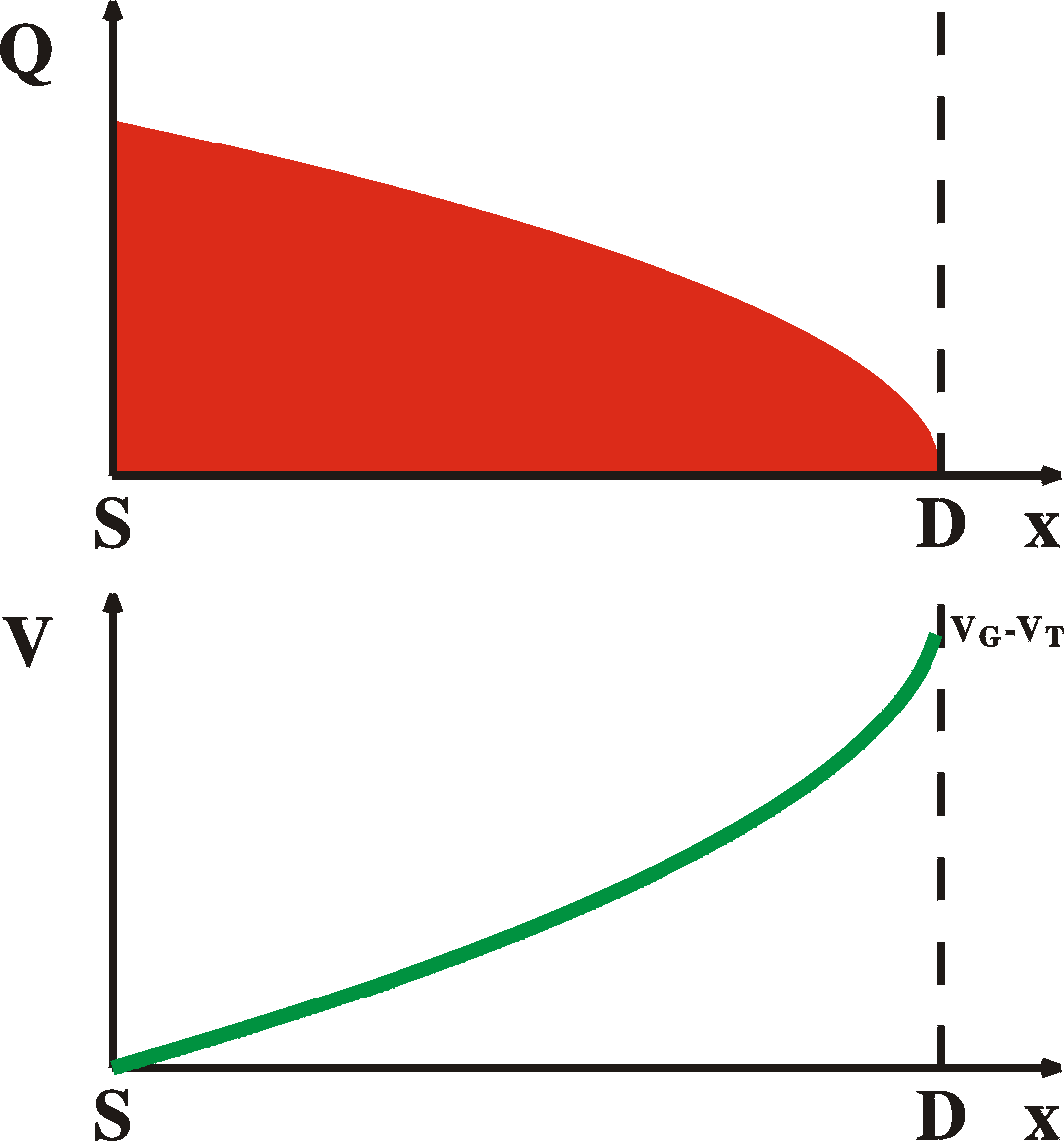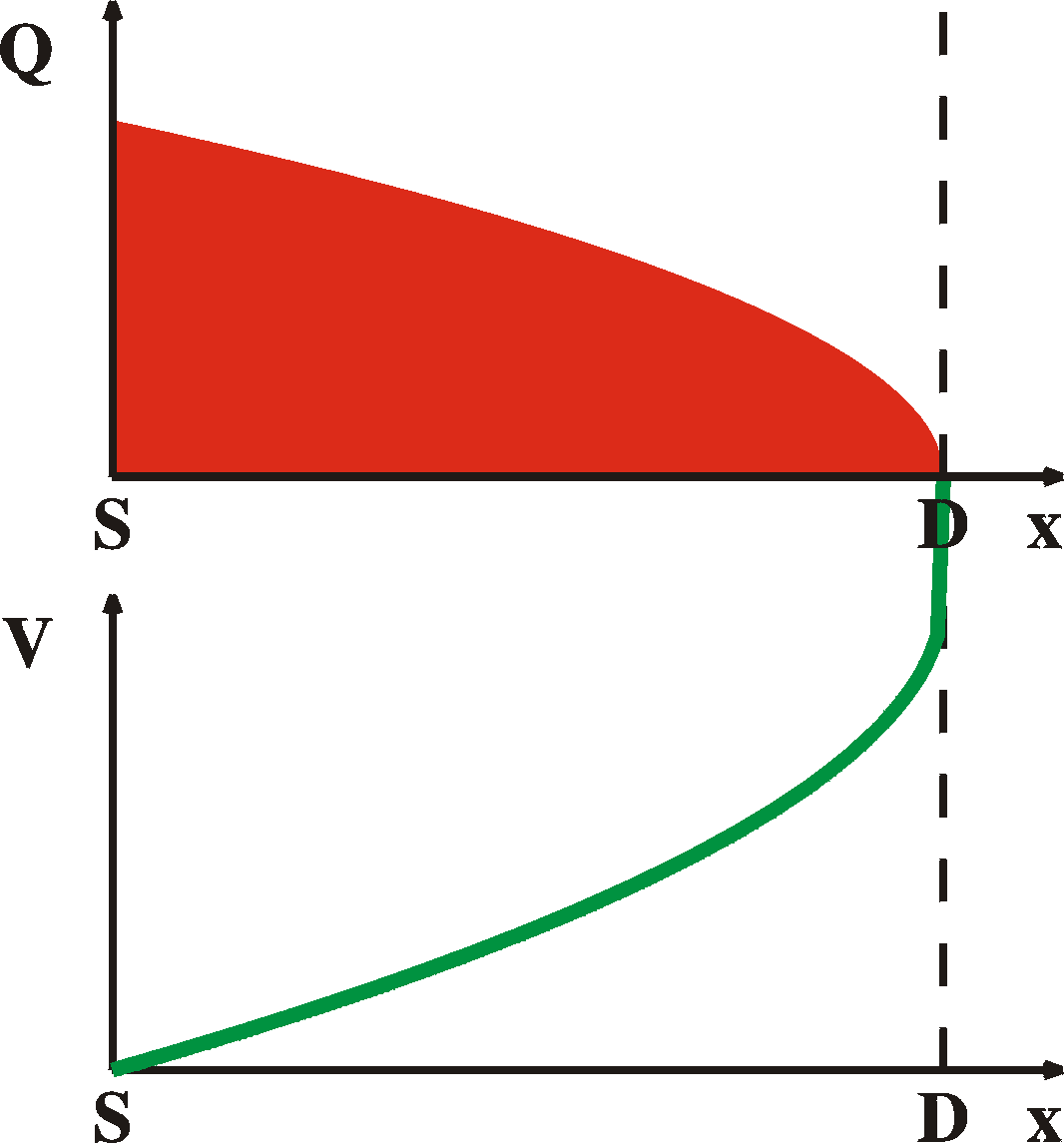Vds = 0 V Vds < Vg LIN Vds = Vg onset of SAT Vds > Vg SAT
Charge distribution and local potential along the channel in a TFT. Note again that the charge in all cases is in a thin layer and there is no "pinch" off where the channel gets zero width. Instead, in the ultra-thin layer, the charge density goes to zero. In all cases the channel is completely accommodated within the first monolayer of the active layer.

### Linear region

In the linear region (Vds approaching zero), the density of charge and the electric field is homogeneous across the channel. In that case, the above equation reduces to
 Ids    = m CoxVgVds W/L
This allows for the definition of the as measured field-effect mobility as
mFET
=
 dIds 1 dVg CoxVds W/L

For reasons described later, the as-measured mobility can be substantially lower than the intrinsic mobility m
An expression can also be derived for the field-effect mobility in saturation, but because the saturation behavior is often far from ideal and it is therefore not advisable to use the saturation region for the determination of the as-measured mobility.

### Threshold voltage of a TFT

For pure, intrinsic materials, the relation between free-charge and bias is linear. Therefore, the threshold voltage in these transistors is zero.

 VT   =  0
The threshold voltage can deviate from zero in two ways:
• For materials with traps, some of the charge in the active layer can have zero mobility. While still contributing to the charge-bias relation (Eq. 1), it does no longer contribute to current (Eq. 2). In some cases, as will be shown, this can be modeled by a threshold voltage and this voltage has the same sign as the bias when the channel is present. (For instance, VT is negative for a hole-channel TFT)
• For conductive materials (for instance doped semiconductors) there can be free charge in the channel even without a bias, resulting in so-called "normally-on" devices. A threshold voltage needs to be applied to the gate to close the channel. In other words, the threshold voltage has opposite sign compared to the gate bias in normal operation. For instance, for a hole-channel TFT the threshold voltage is positive.
These example will be discussed in more detail later,

### Sub-threshold current

For MOS-FETs it can be shown that the sub-threshold current grows exponentially with the gate-bias and has the dopant concentration as an important parameter. In TFTs, as demonstrated above, the threshold voltage is zero. It can also be said that for an ideal TFT the sub-threshold current is zero. This is caused by the fact that the starting material is assumed to have zero carrier density and all the free carriers are induced by the gate. There are reasons why, for real materials this is not an adequate description
• For semiconductors with reduced electronic band gap or at high temperatures, the residual free-carrier density is not negligible, ni2 = NVNCexp(-Eg/kT).
• For doped semiconductors a high density of free carriers exists because, even for low temperatures, most dopants are ionized.
These two types of semiconductors will result in "normally-on" devices with a non-zero threshold voltage and a sub-threshold current. They will be discussed later.

### Leakage current in a TFT

 In many cases, the insulator is less-than-perfect and currents may exist through the insulator to the gate. In a very rudimentary way, we can simulate this by placing a resistor bridging the drain and the source (assuming the current meter is placed at the drain), as shown here on the picture on the right. A better simulation would have at every point in space (x) a resistor bridging the channel and the gate, but for the moment these pictures are informative enough, I hope. In the IV curves (left), the curves cross (in the linear region) at Vds = 1/(aRdg), Ids = 1/(2aRdg2) with a = m Cox W/L Assuming this simle model of a shunt resistor Rdg, it is easily understood that, to circumnvent measuring the leakage current and focus on the channel current, a good way is to measure the locust curve, (Ids as a function of Vds = Vg). In principle, leakage current should then disappear from the measurements.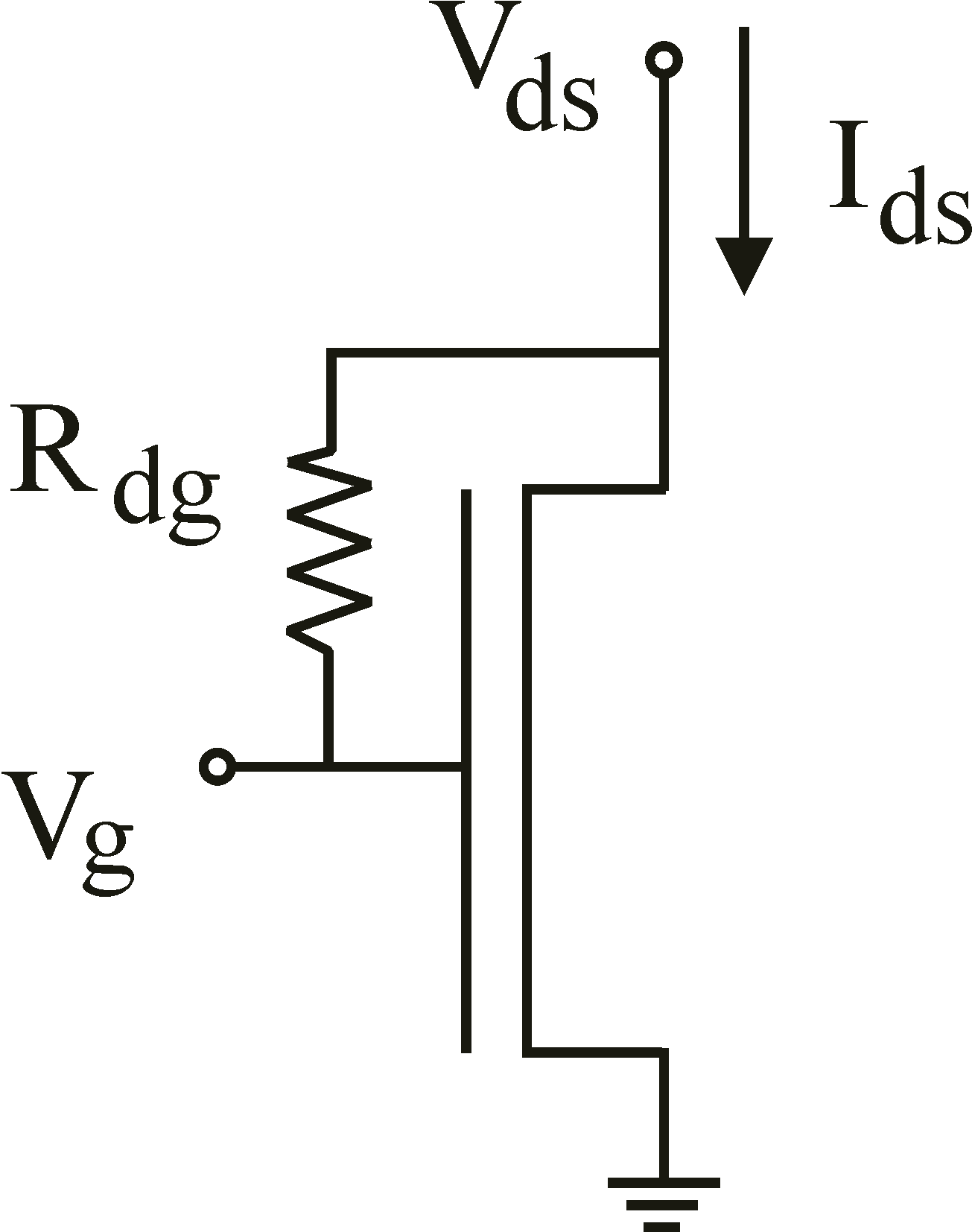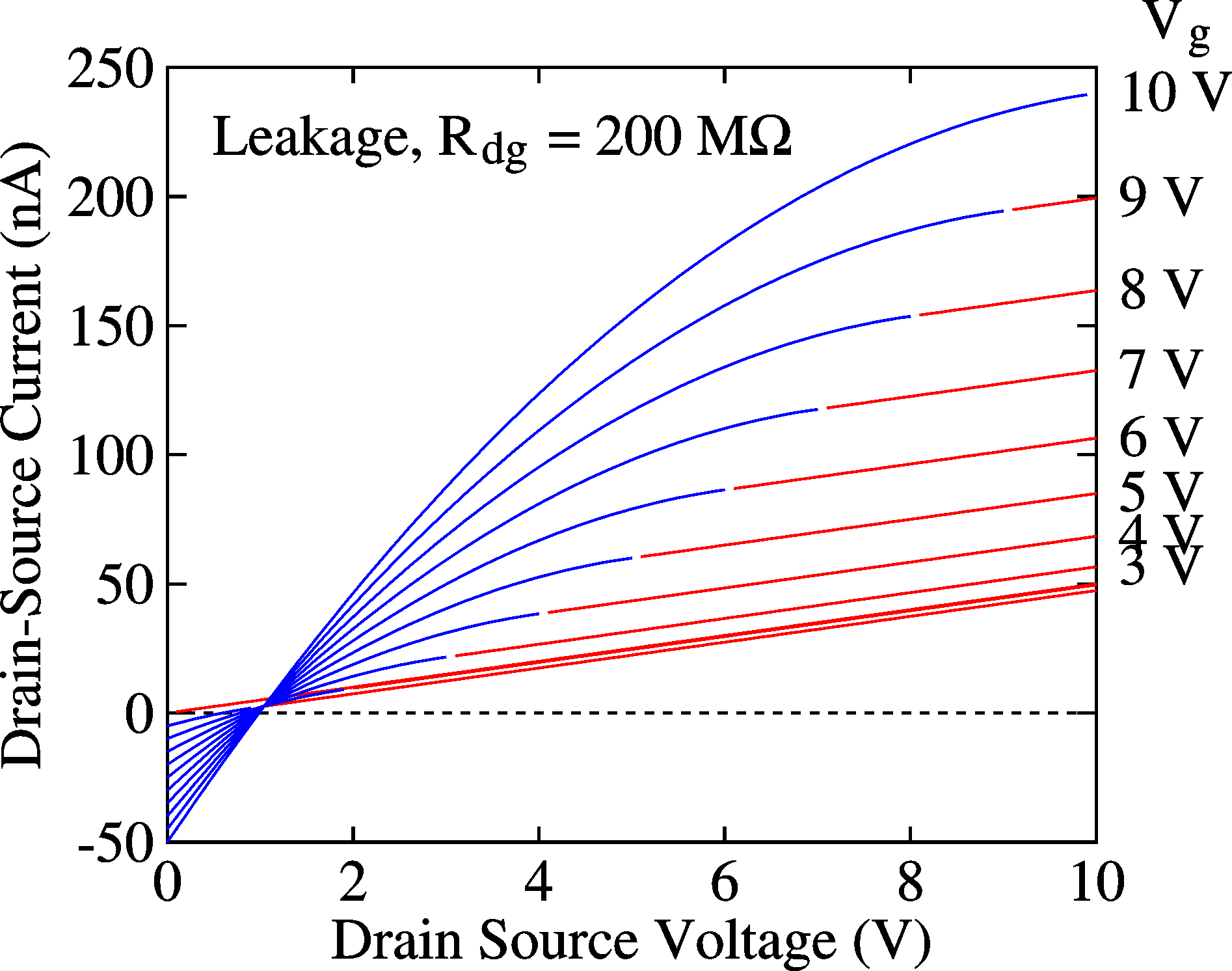Simulation of leakage current in a TFT (see circuit above). IV curves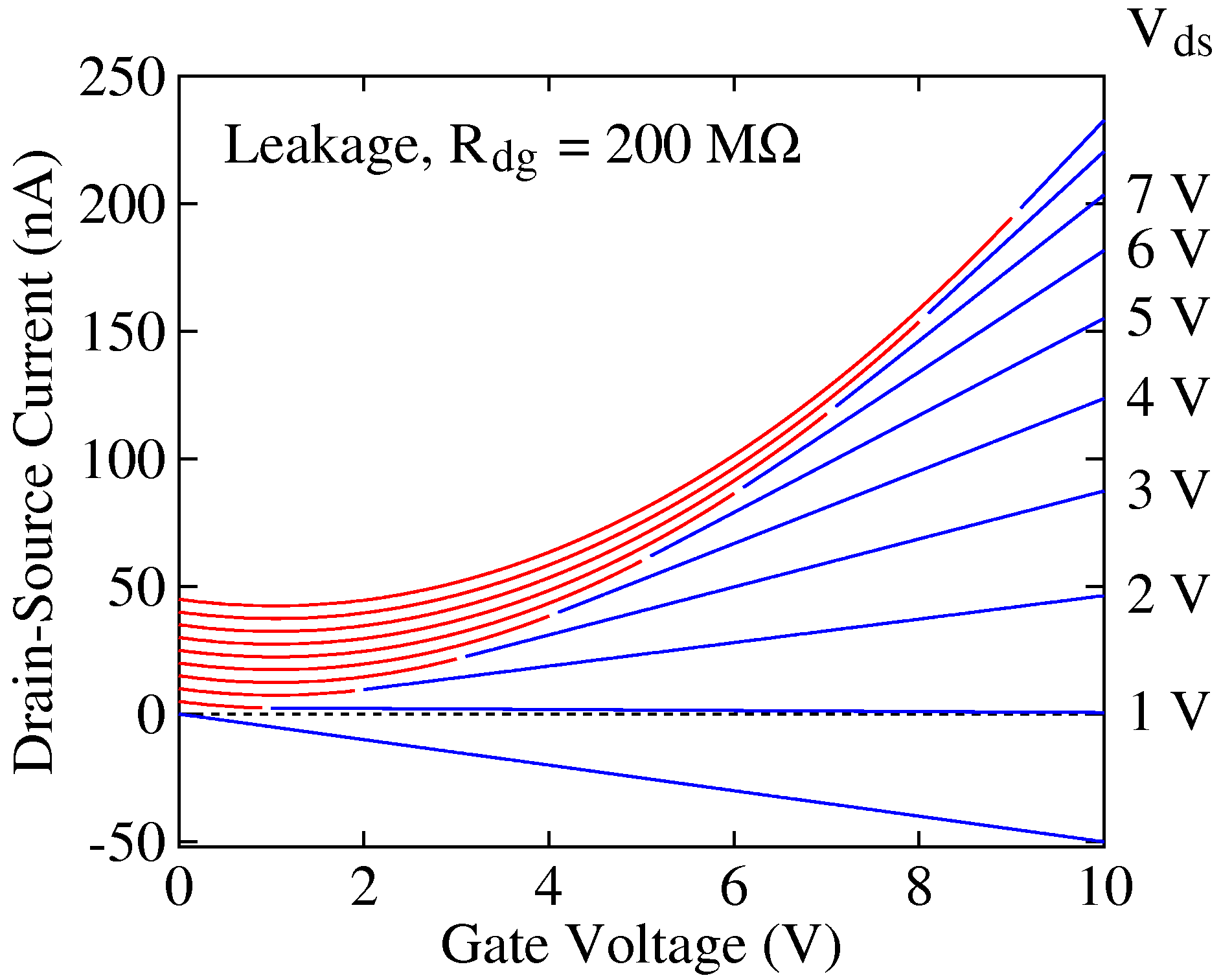Simulation of leakage current in a TFT (see circuit above). Transfer curves

### Ambipolar materials

 Ambipolar materials are materials which have a high electron and hole mobility and can thus conduct both. Because these electrons and holes can thus be made to meet somewhere in the middle of the channel, far away from the electrodes, they can be made to produce light when they recombine. Such light-emitting field-effect transistors can also be made from two separate layers, one with high electron mobility and one with high hole mobility, but the description here will assume that the material itself is ambipolar. For such materials, saturation cannot occur. The reason of this is quite simple. In unipolar devices, when the density of the holes goes to zero, a large field can exist while maintaining a small current. At this point the opposite carrier (n) gets a high density (because n p = ni2 still holds, as long as the currents are low) but because they have low mobility, they cannot contribute to current. On the other hand, in ambipolar materials the mobility is also relatively high and no strong fields can be maintained. In the equation replacing Eq. 2, Ix(x) = - q [p(x) mp +  n(x) mn] W dV(x)/dx which reduces to the original Equation 2 when mn = 0. It is obvious that always a hole current or an electron current exists. The figure on the left shows an example of the charge and potential distribution in an ambipolar TFT. Note the separate regions of holes and electrons. At the meeting point, electrons and holes can interdiffuse and recombine radiatively. By playing with the gate and drain voltages the position of the cross-over point can be programmed, as well as the total current (and thus light intensity). See Synthetic Metals 156, 1305 (2006)and Optica Applicata 36, 373 (2006).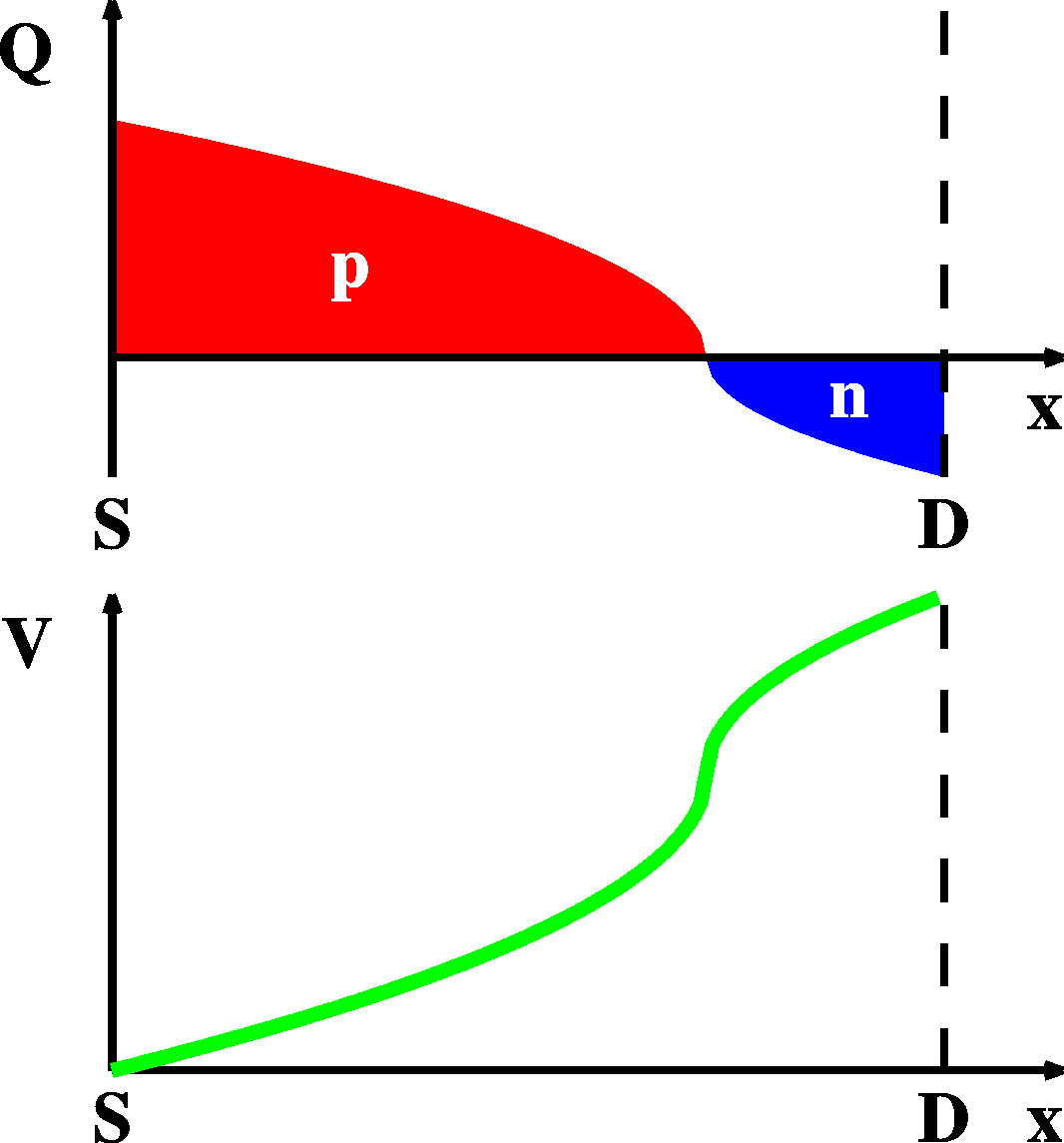Charge (Q) and potential (V) distribution in an ambipolar TFT.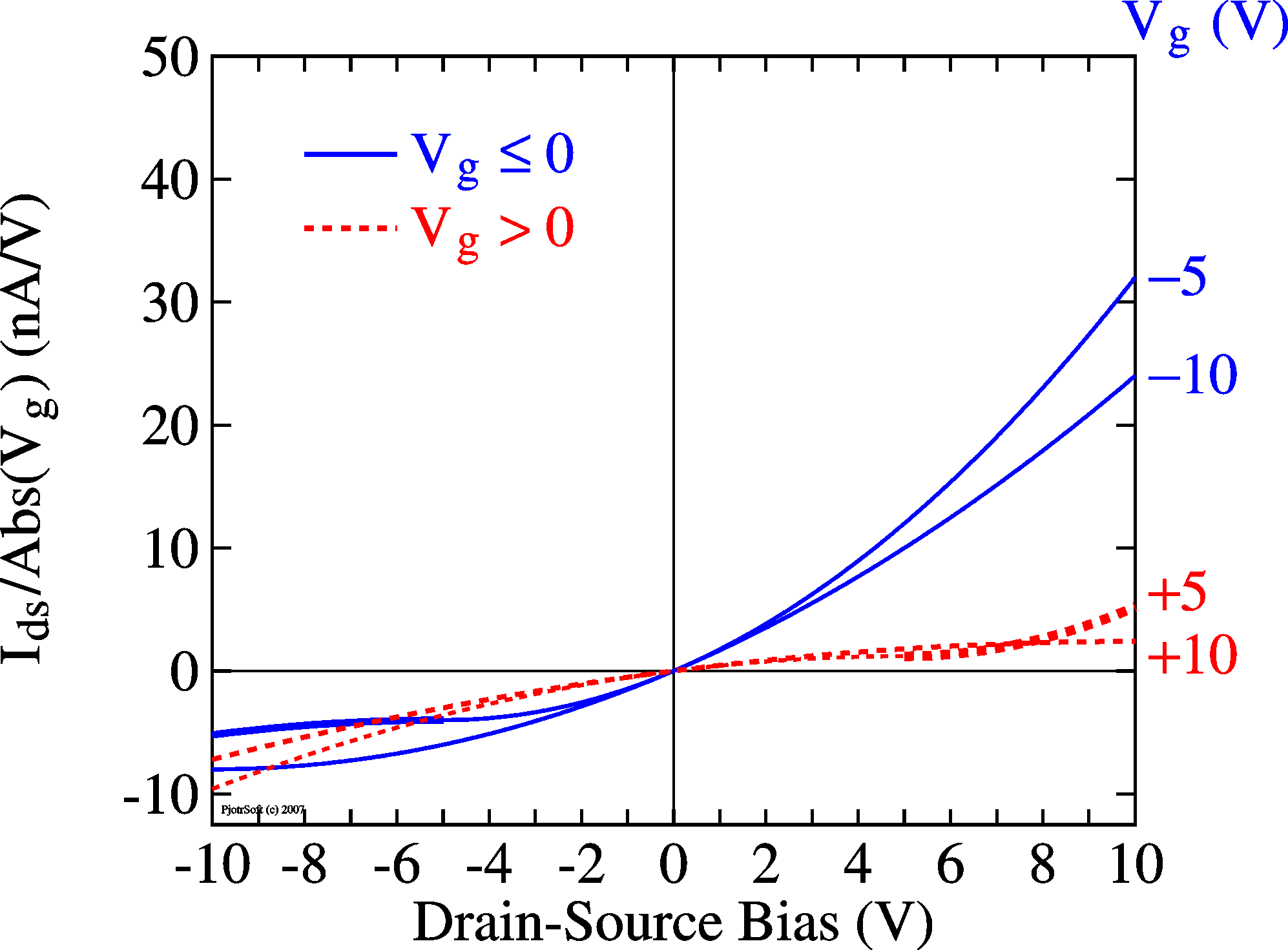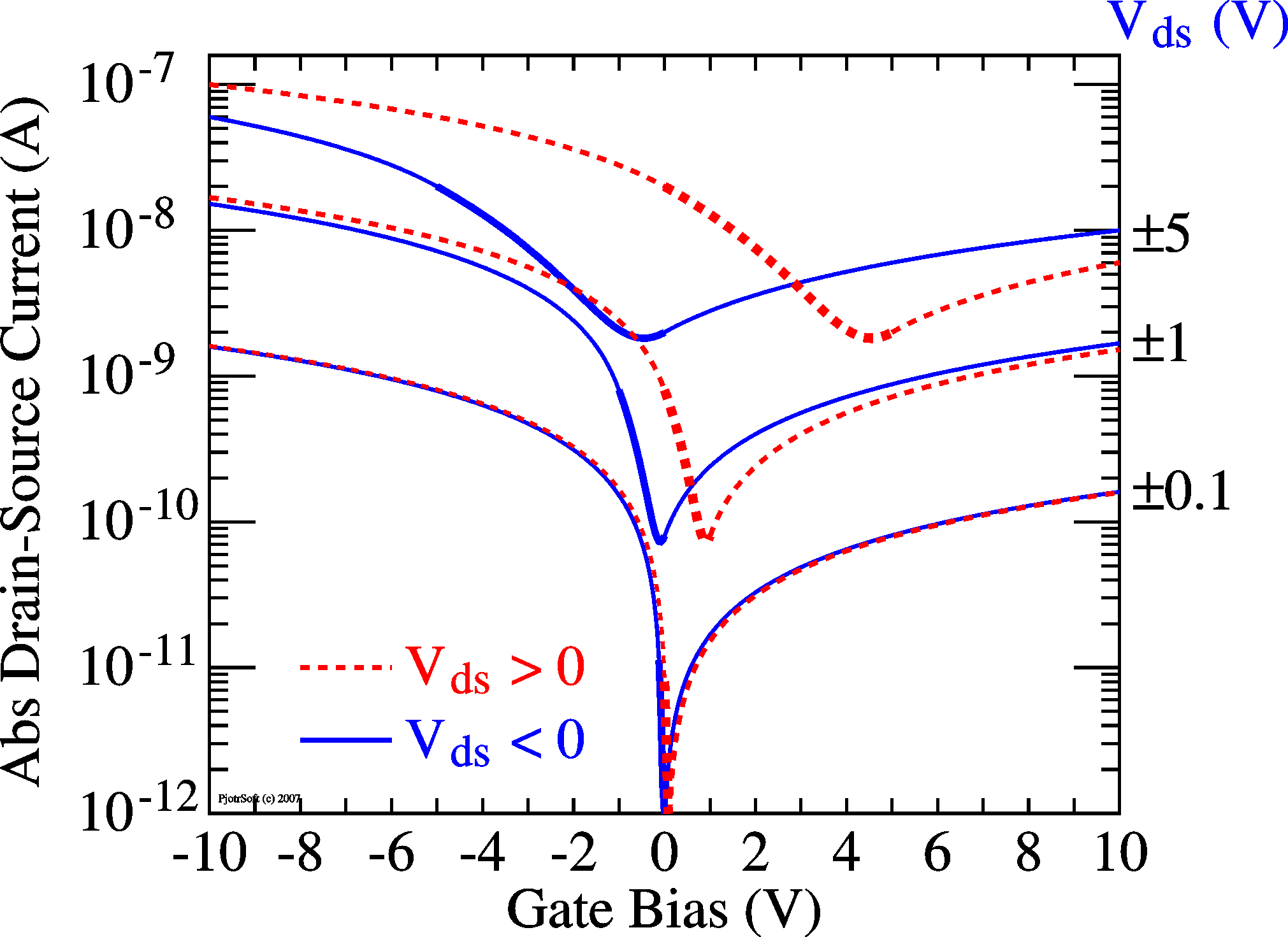Ambipolar TFT IV (output) curves in linear scale (left) and log scale (middle). Right: transfer curves. mp = 10-4 cm2/Vs. mn = 0.1 mp (middle and right) or 0.3 mp (left)

## Metal-insulator-metal TFT

Advanced Materials 20, 1 (2008)## Contact resistance in TFTs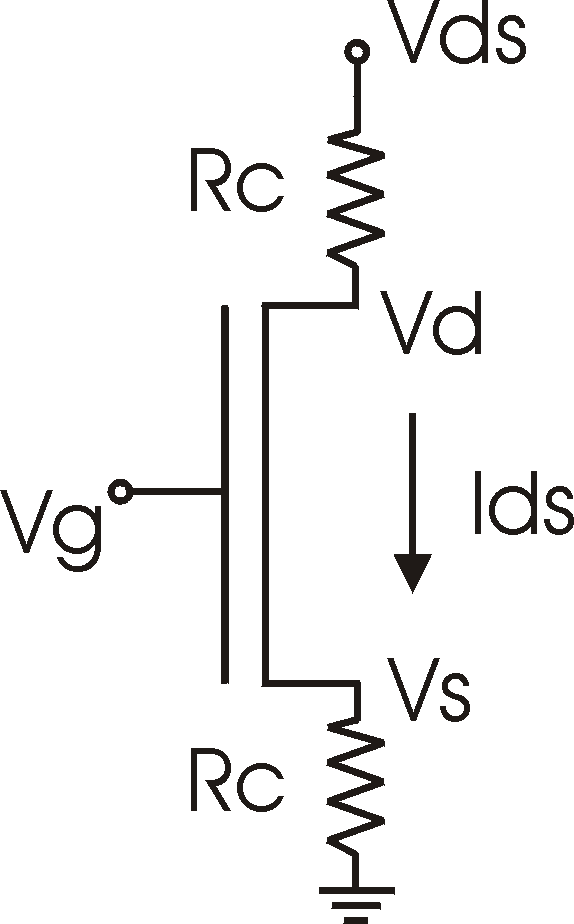Vs = IdsRc   Vd = Vds - Ids Rc   Vgs = Vg-Vs   Ids = mCox (W/L) Vgs(Vd -Vs ) In the above discussion it was assumed that the injection of carriers is not a limiting factor. In other words the contacts were considered low-ohmic However, the contacts can cause a severe barriers for the injection of carriers. The most obvious one is when at the electrodes a high-resistive region is formed. This causes the current to grow sub-linearly in the linear region. This can easily be shown in a simulation. For strong currents, the contact resistance can become the limiting factor and the current saturates and becomes independent of Vg: We have to imagine that the FET is made up of two contact resistances (2Rc) and the channel resistance, connected in series. Initially the current grows linear with VG (as explained by the text above). The channel resistance is thus proportional to 1/Vg.For large Vg the channel resistance disappears and the current settles at Vds / 2Rc. More exact: when the current is increasing, the contact resistance induces a voltage drop at the source, Vs = IdsRc, the field at the source (Vgs) is reduced and the current drops (external observables in bold):
SimulationTransfer curves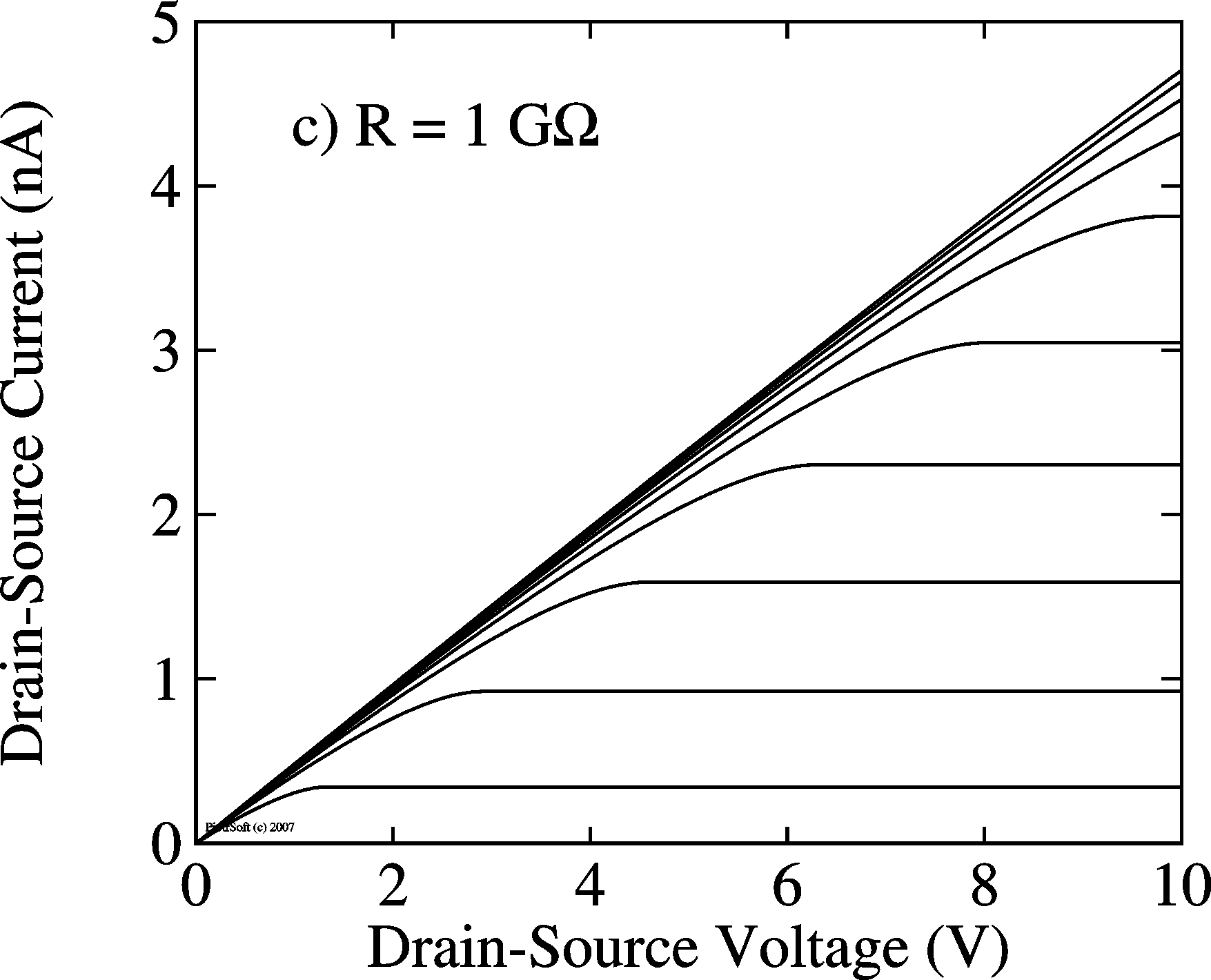IV Curves

## Contact Schottky barriers in TFTs

 The interface regions are not necessarily ohmic, as described above. Contacts are normally made of metal and the contact of this metal with a semiconductor might result in a Schottky diode. When a depletion layer is formed between the electrodes and the semiconductor, the current, might be limited by this Schottky barrier, see the bipolar device theory pages. When such Schottky barriers exist, they come in pairs, with a forward biased Schottky diode at the drain and a reverse-biased Schottky diode at the source (or vice verse). In other words, the maximum current through the device is a reverse-biased Schottky barrier current. When the Schottky barriers are the limiting elements, the current thus follows a hyperbolic tangent, Ids = tanh(Vds) and saturates at a voltage of approximately Vsat = kT/q = 26 mV at room temperature, see the simulation below. This is not what is normally observed for TFTs. We conclude therefore that Schottky barriers play no role in TFTs. Synthetic Metals 156, 1305 (2006).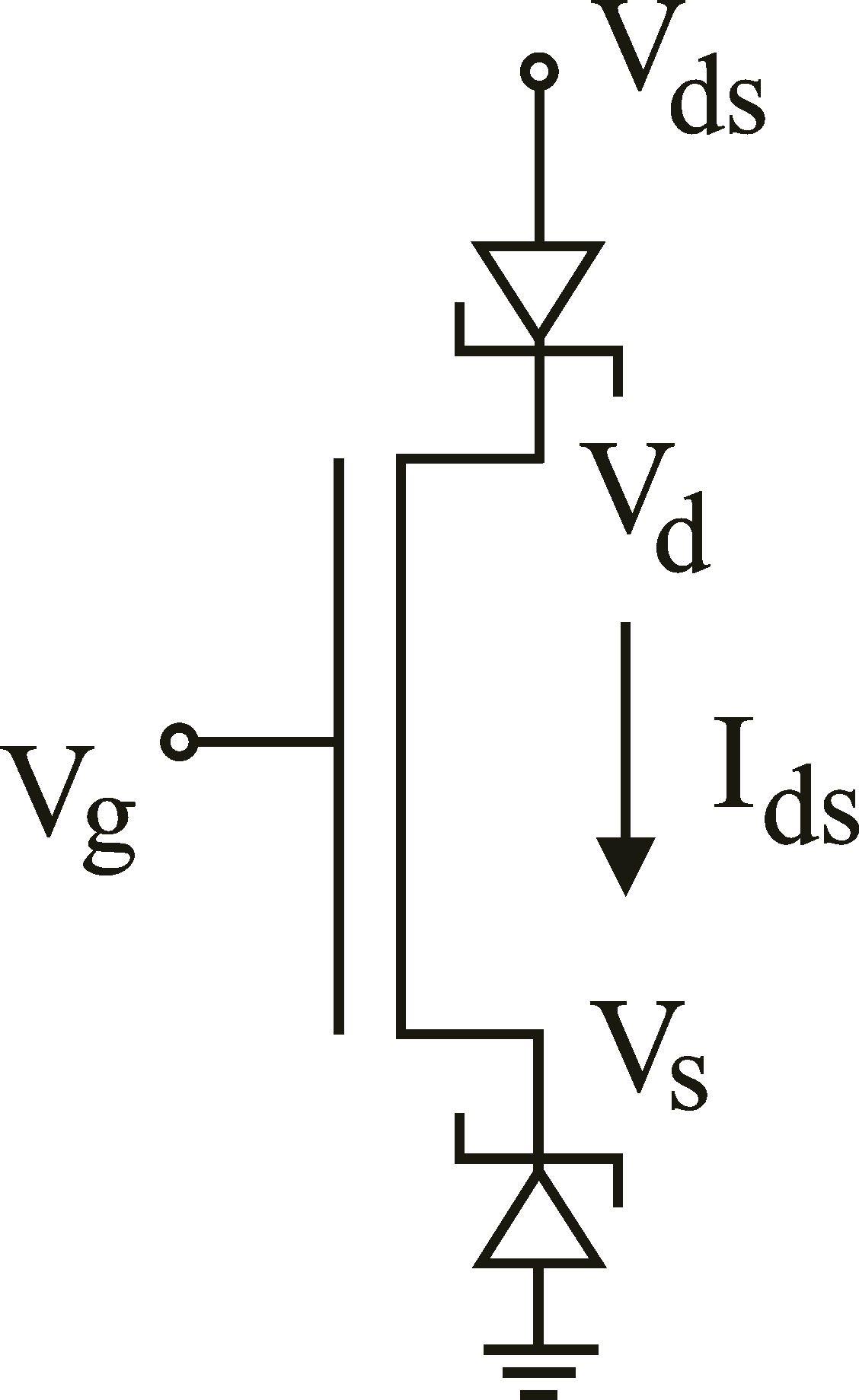Equivalent circuit of an FET  with Schottky barriers at the contacts.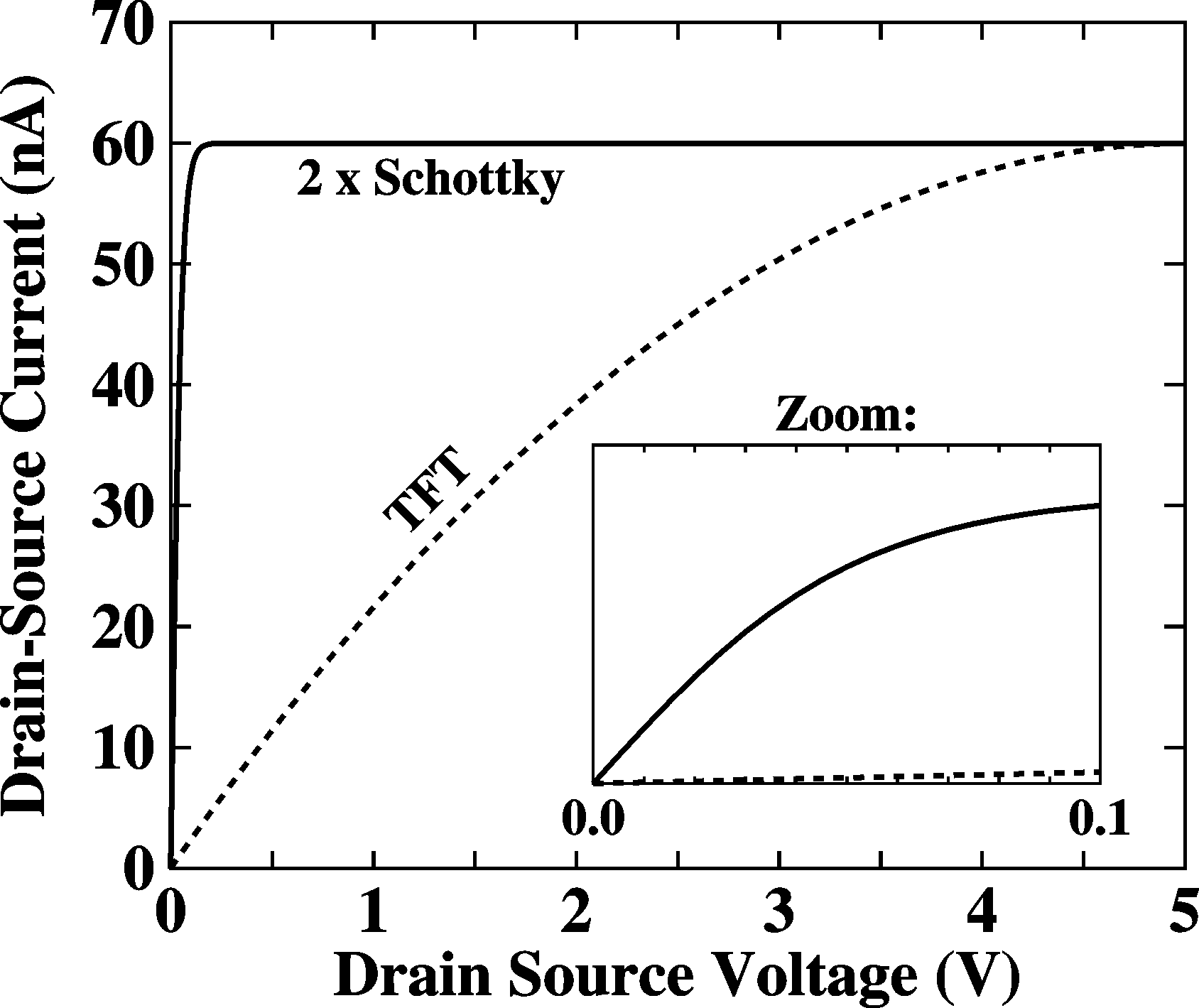Simulation of an FET with Schottky barriers at the contacts as given on the left.

## Description of metal contacts in TFTs

There is a more fundamental reason why analyzing TFTs with Schottky barriers does not make sense and that is that the symmetry of the TFT prohibits such analysis.
A Schottky barrier analysis is used in so-called Schottky diodes. In this case, the interface between the metal and the semiconductor is perpendicular to the current and is so large as to be considered infinite for the sake of the calculations, where the rel area of the interface only enters as  a scaling parameter in the final current-voltage relation. In other words, the symmetry of the interface is to have all physical quantities independent of y and z and only depending on x, the direction of current. For this specific symmetry, the Maxwell Equation, namely "divergence of displacement is equal to charge density", reduces to the famous Poisson Equation, namely "double space derivative of potential is charge density divided by epsilon."

d2V(x)/dx2 = r(x)/e

However, the symmetry of a thin-film transistor metal-semiconductor interface is far from having this symmetry. Quite to the contrary; the direction y (perpendicular to the surface and to the current) is very asymmetric, with in fact having only a thickness of one mono-layer. Even more important, the close presence of the gate totally destroys the symmetry and is of fundamental importance (by definition) in TFTs. Ignoring the gate in the analysis of the source-drain contacts is an error.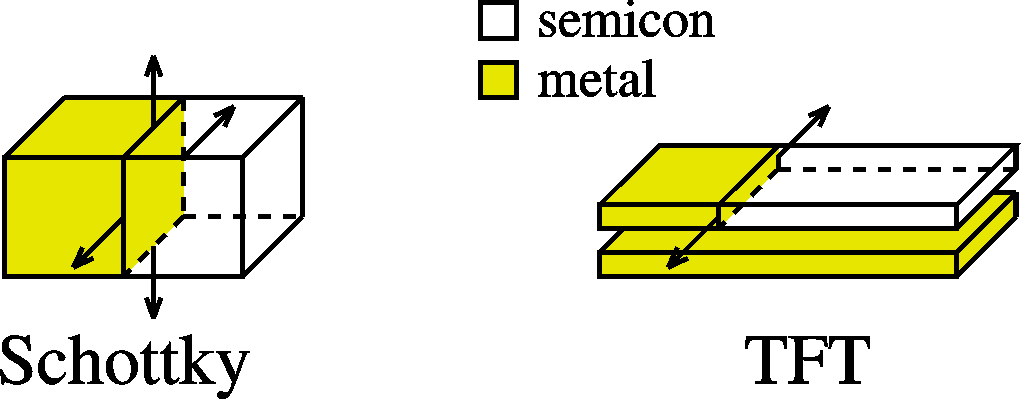We thus reason differently and to describe the effects of the contacts, we continue to hold on to the charge-potential relation of Eq. 1. Then it can easily be shown that the effects of the contacts are minimal. The reasoning is as follows: If a current is measured, this implies free charge (Eq. 2). Free charge means that the Fermi level is somewhat close to the levels responsible for conduction. In the metal the Fermi level is defined by the top of the sea of electrons. The difference between the Fermi level in the metal and the semiconductor is the height of the barrier. We thus argue that for measurable currents, this barrier is less than 100 meV and negligible. Moreover, we predict a gate-bias-dependent barrier-height because the carrier density and thus depth of the Fermi level is bias dependent. Interesting in this respect is the observation of exactly such a behavior by Buergi et al. and Yagi et al.metal-semiconductor-metal TFT system before contactmetal-semiconductor-metal TFT system after contact

This has been described in Organic Electronics 8, 300 (2007).

## Effects of traps on the electrical characteristics of TFTs

Traps are localized electronic states that can capture free carriers. For instance, a hole trap can capture a hole in a reaction T + h --> T+, and the resulting positive charge becomes immobile and unavailable for conduction. It is obvious that they have a mostly detrimental effect on the performance of the device, be it a TFT or a diode and their study therefore of utmost importance.
The effects of traps on the electronic properties of TFTs are manifold:
• Reduced mobility; Poole-Frenkel
• Temperature dependence of mobility
• Non-linearities in IV curves
• Non linearities in transfer curves; power-law, Ids ~ Vgn)
• Meyer-Neldel rule; activation energy of current and mobility depends on bias
• Transients; Ids depends on time, often following multi-exponentials, resulting in so-called stretched exponential, Ids ~ exp[ - (t/t)b ]
These effects are described in Organic Electronics 7, 592 (2006)(Non-linearities in IV curves, see Synthetic Metals 156, 1316 (2006)). They will be summarized here:

Non-linear IV (output) curves
As shown by Poole and Frenkel, for a huge density of traps, the mobility becomes temperature and field dependent. The field meant here is the field in the direction of the current. A field will put the coulombic potential "on a slope" and the charges can more easily escape the potential well on one side. Poole and Frenkel (see book of Sze) have shown that the effective mobility thus becomes field and temperature dependent. This mobility m(Ex), depending on Ex and thus on x, has to be substituted in Eq. (2) and a differential equation results that is not analytically solvable. The figures below show simulations made in MatLab. For more details, see Synthetic Metals 156, 1316 (2006).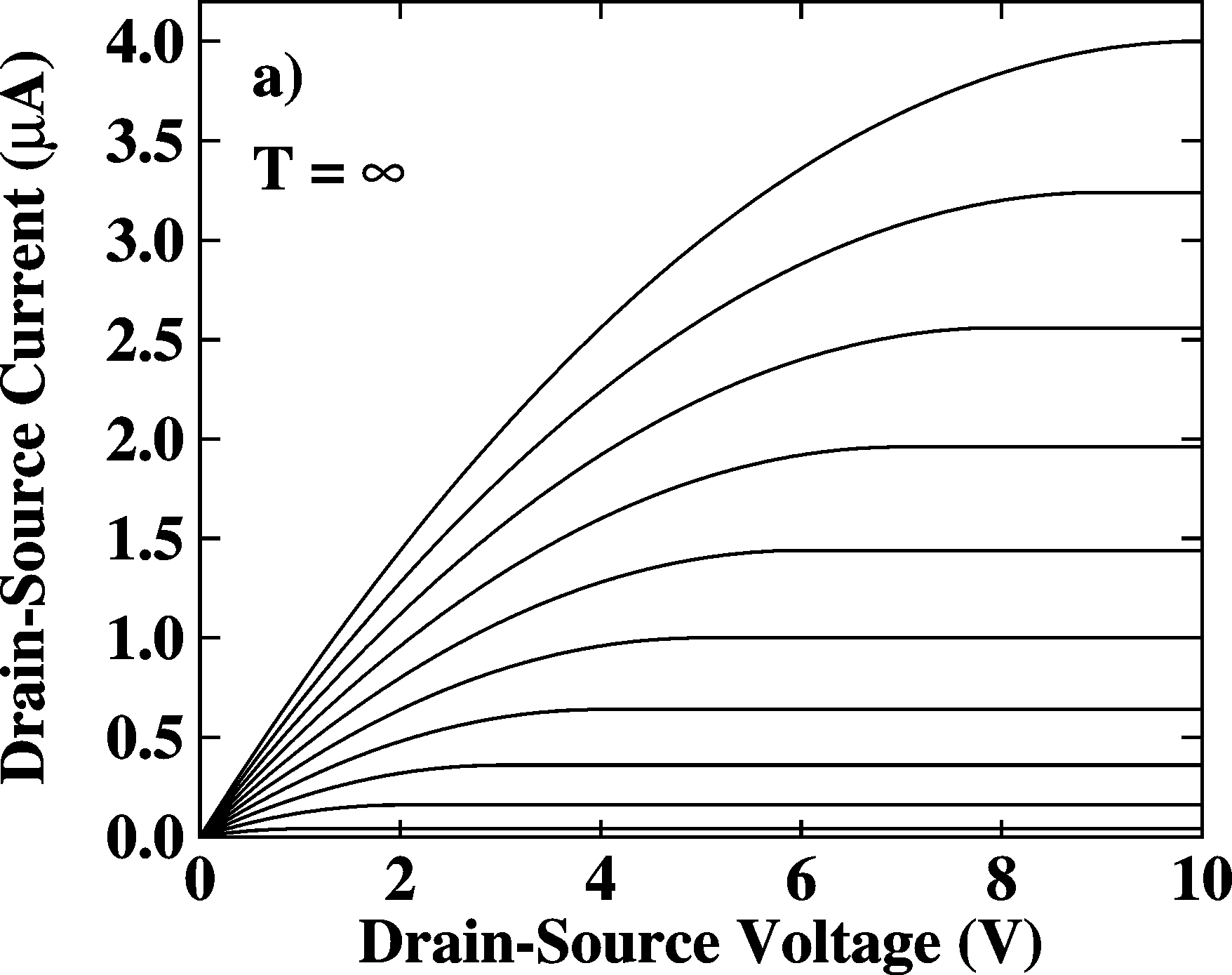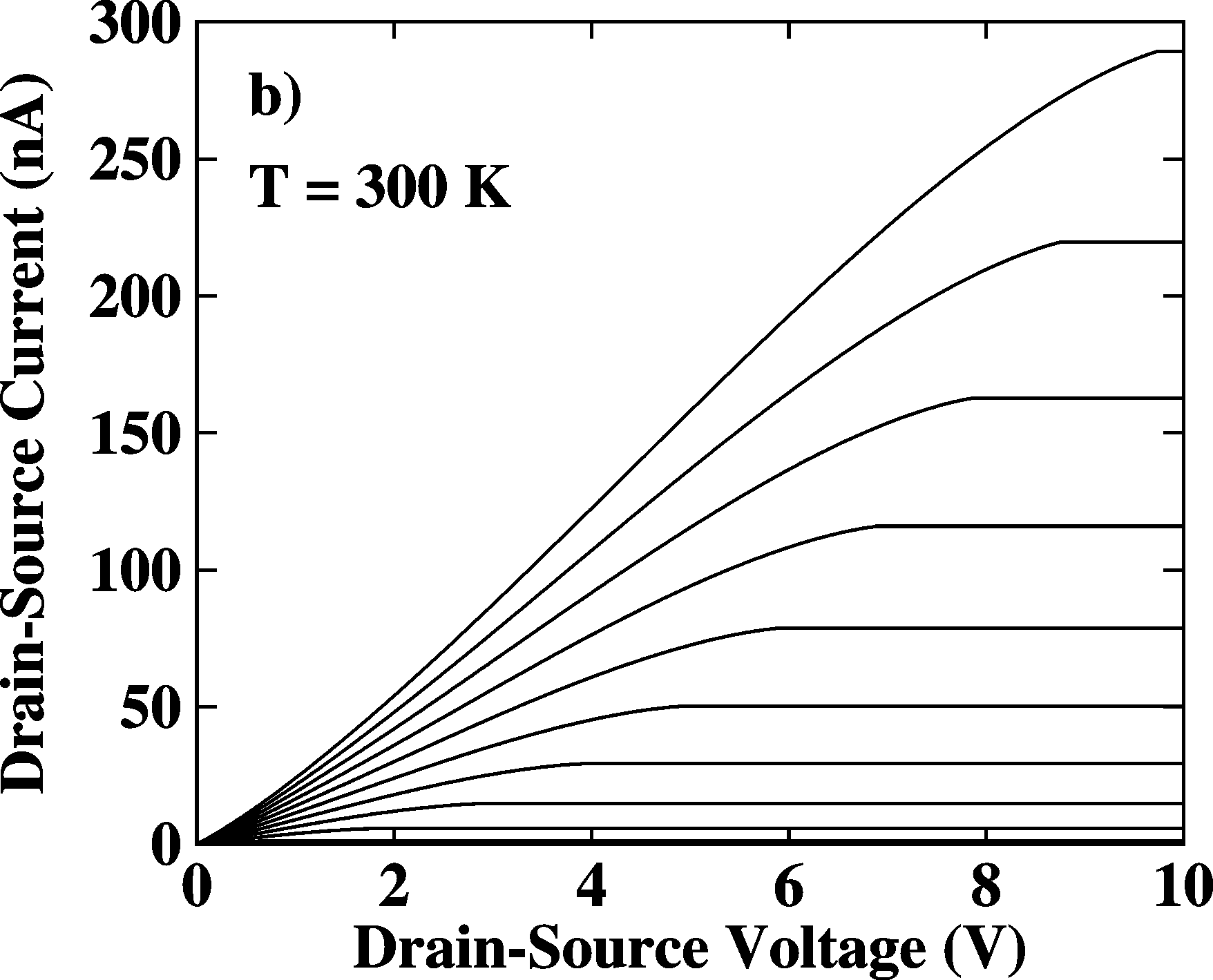IV curves of a TFT with a huge density of traps according to the Poole-Frenkel model. The peculiar sharp bending at lower temperatures is caused by the fact that no diffusion currents were included in the model.

Non-linearities in transfer curves (Temperature and bias dependence of mobility; the Meyer-Neldel Rule)
Traps can distort the transfer curves (instead of linear become power-law, Ids ~ Vgn) and make the mobility temperature and bias dependent. See Organic Electronics 7, 592 (2006). The results are summarized below: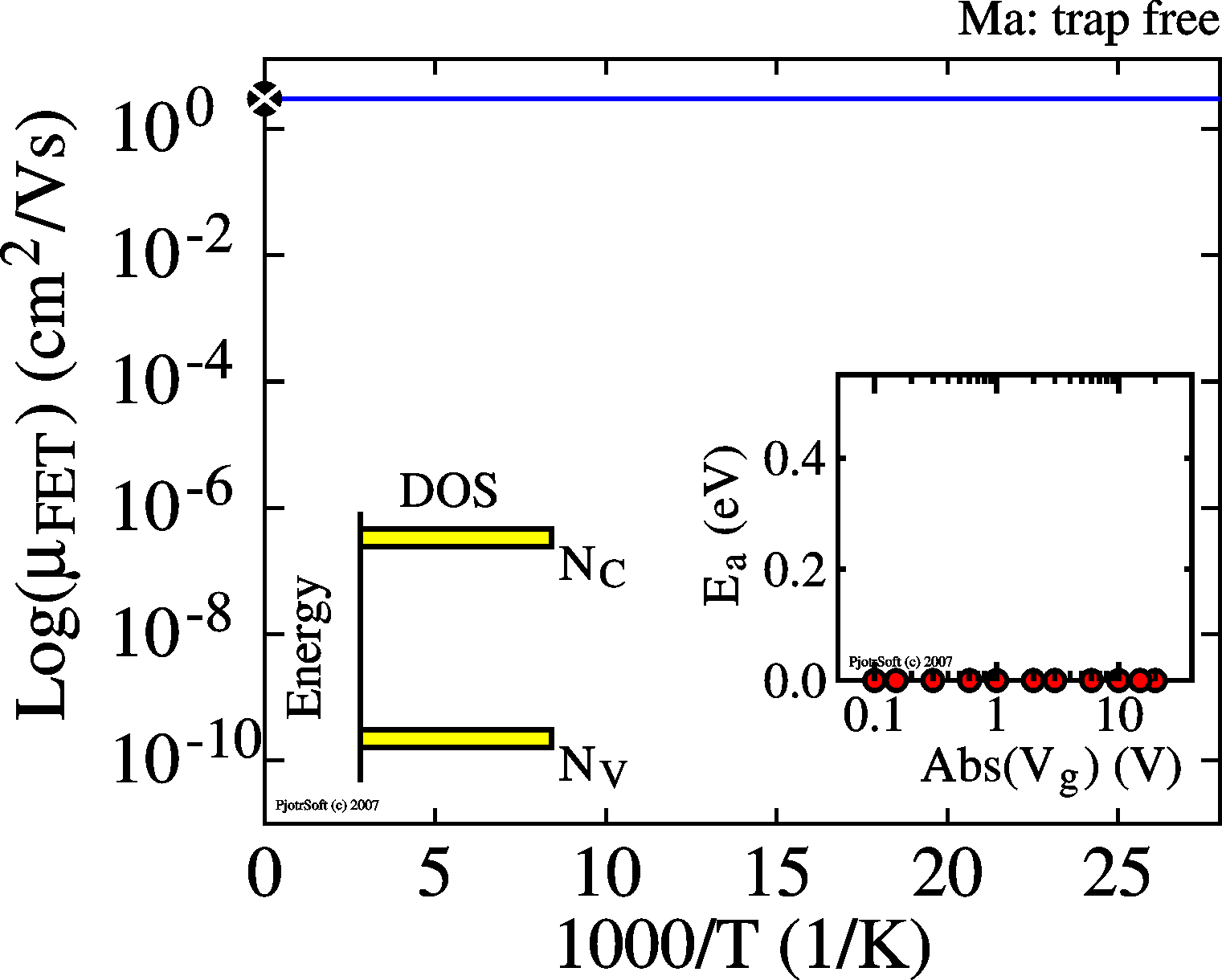Trap free device. The (as-measured) mobility is constant - independent of temperature (the Arrhenius plots are horizontal lines; EA = 0) - independent of bias (the activation energies are 0 for all Vg, see inset) The IV and transfer curves are as described above in "the basic model".With a huge density of discrete traps, a substantially amount of the charge induced by the gate bias is on the traps and doesn't contribute to current. In thermal equilibrium the ratio of free (p) to trapped (pT) charge follows a Fermi-Dirac distribution. For huge densities of traps this can be approximated by a Boltzmann distribution. Thus p/pT = (NV/NT) * exp(-ET/2kT) with NT the density of trap states and NV the desnity of conductive states. The total charge induced by the gate is the sum of trapped and mobile charge (in equilibrium). The current is proportional to p. The result is that both the current and the mobility become temperature dependent with the activation energy found in an Arrhenius plot equal to half the trap depth ET, independent of Vg. However, The transfer curves look normal because the mobility is reduced and depends on temperature, but does not depend on bias. When the mobility also depends on the in-plane electrical field (what is normally the case), the IV curves become distorted (see above, "non-linear IV curves"), otherwise the IV curves are normal.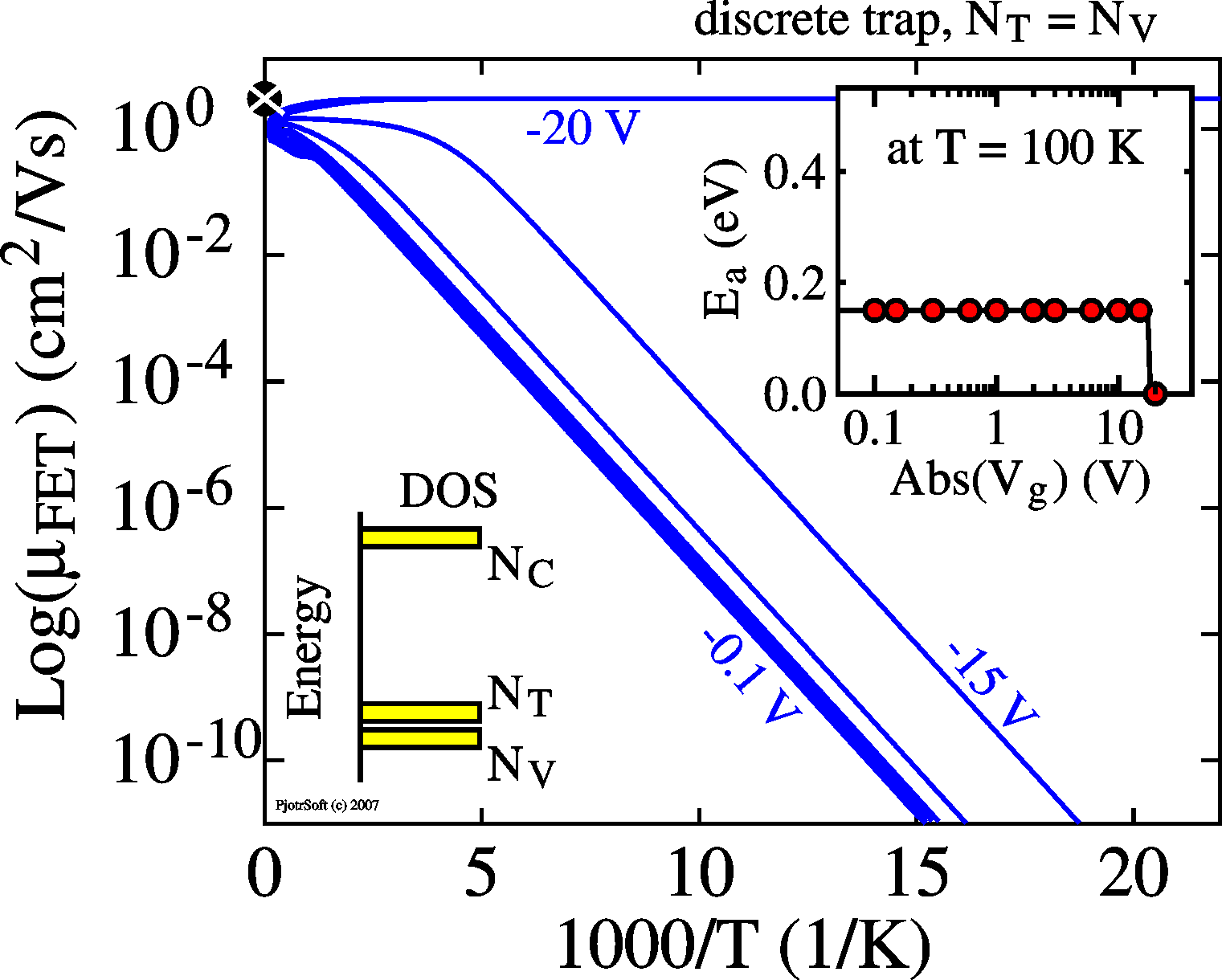When the density of traps is roughly equal to the density of conductive states (for instance valence band) the Arrhnius plot resemble those  above,  up to a certain gate bias Vg. After this bias, the trap-free limit Vtfl, the behavior is as in a trap-free device, with a mobility independent of temperature and bias.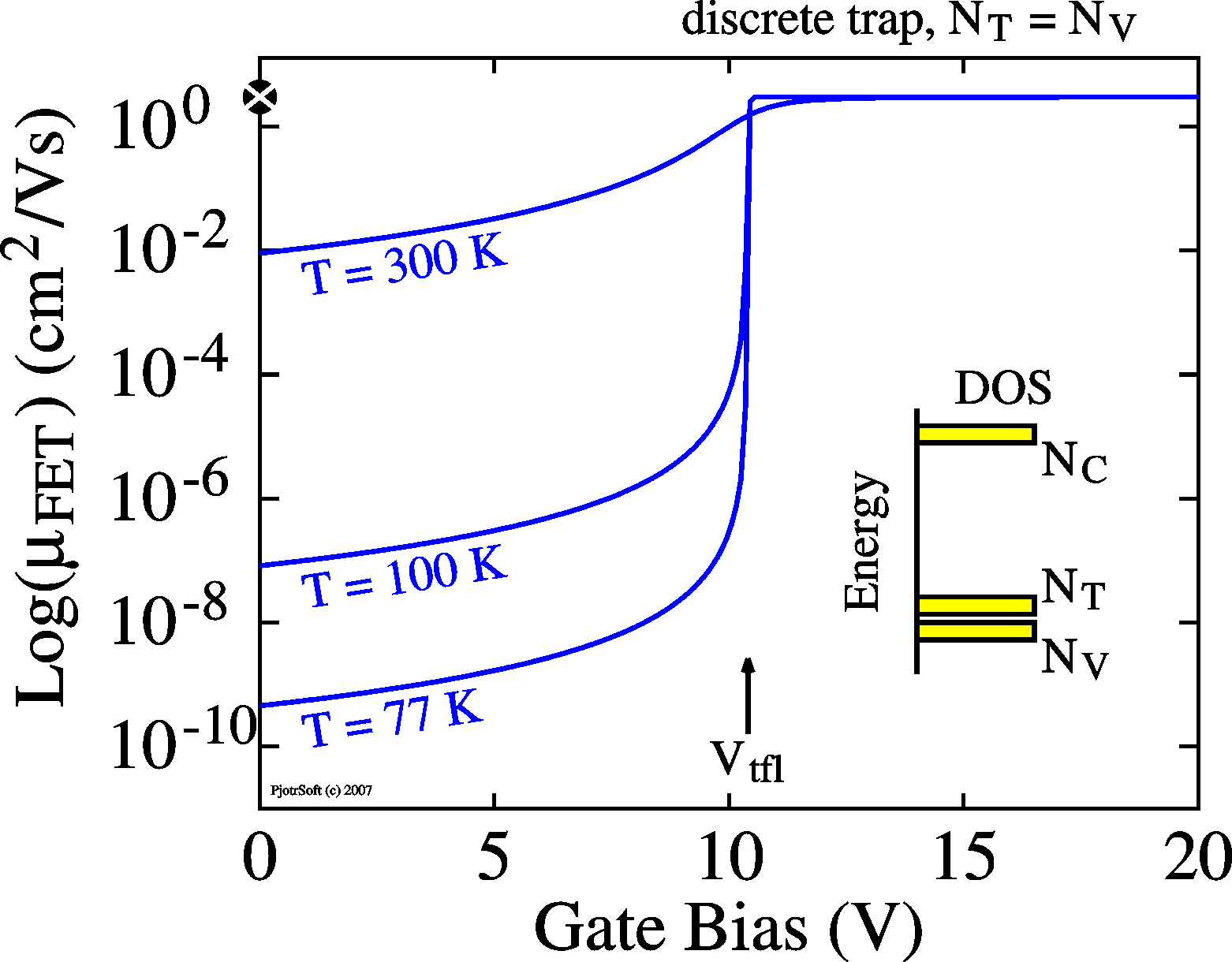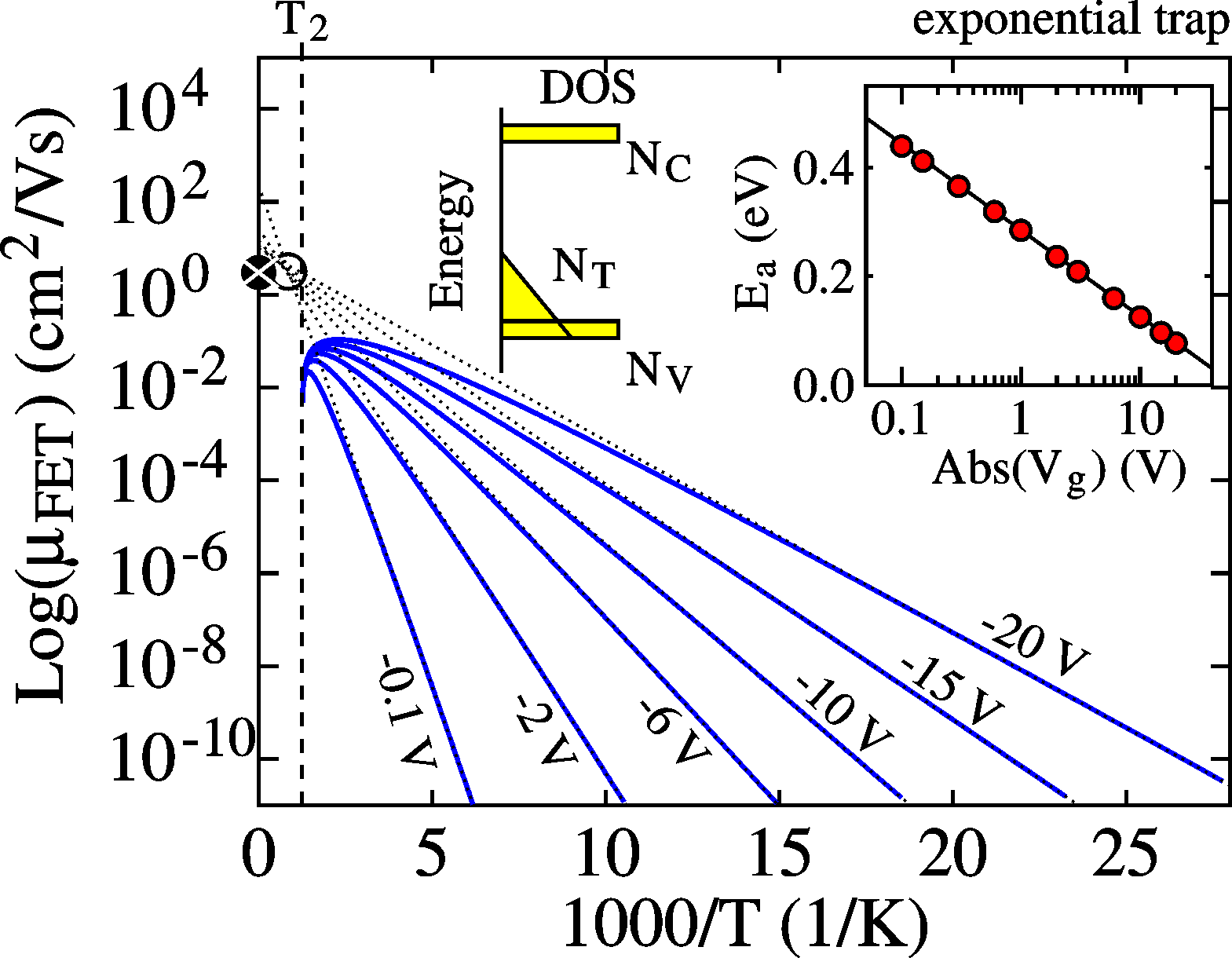For a trap exponentially distributed in energy (DOS schematically shown in yellow), the mobility becomes bias and temperature dependent, resulting in so-called Meyer-Neldel Rule: the Arrhenius plots all point to a single point, the iso-kinetic temperature and mobility.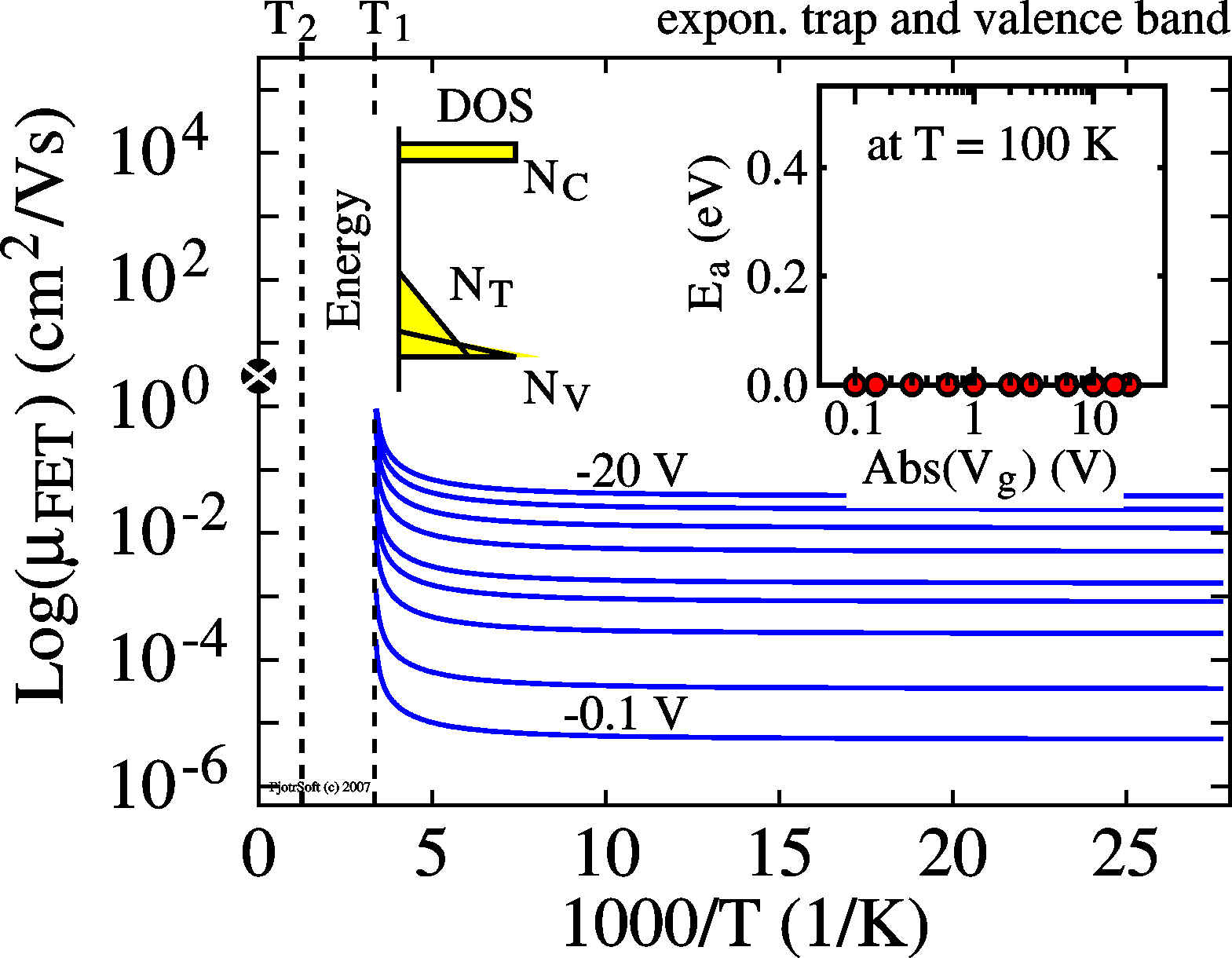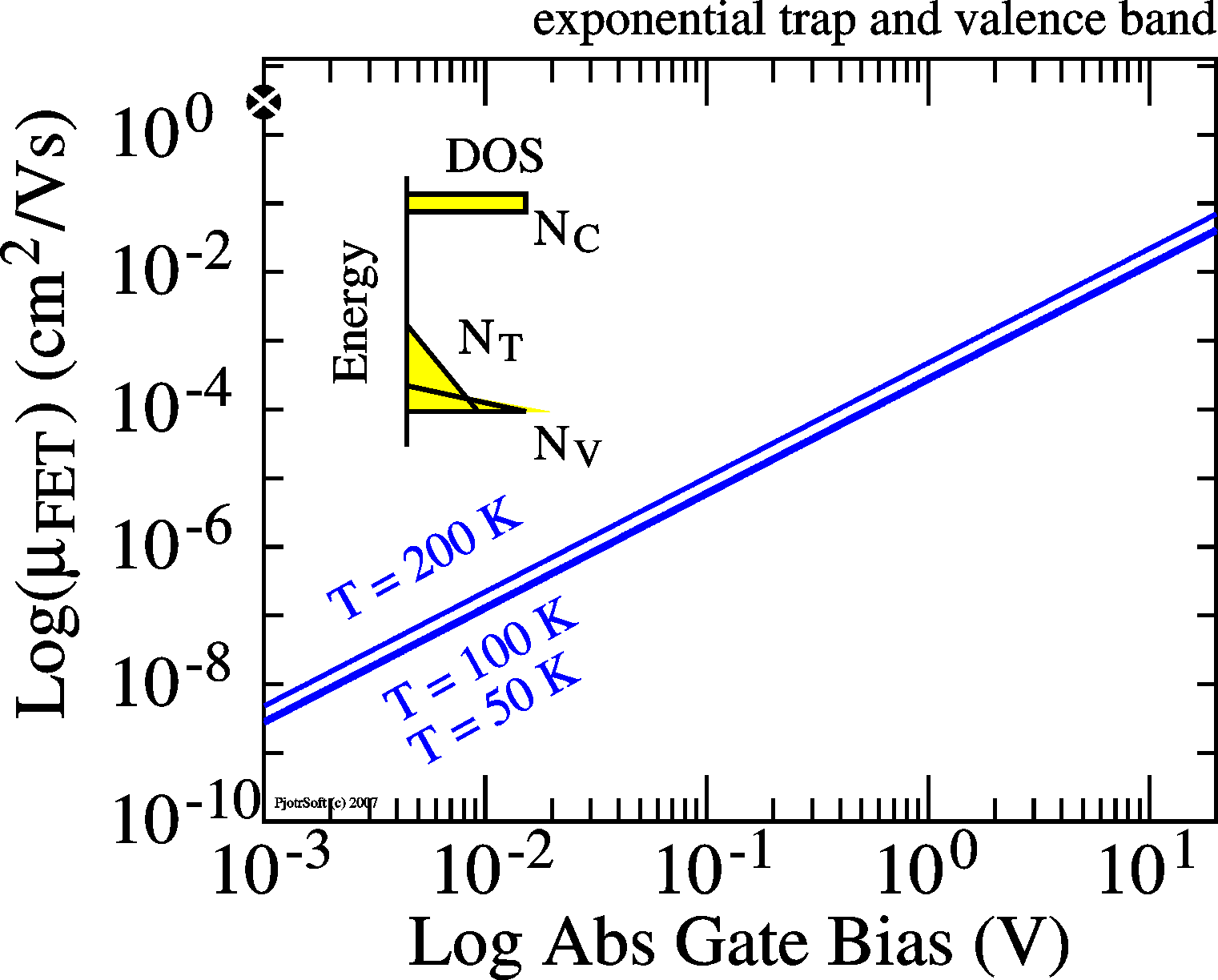When also the conductive states have an exponential distribution in energy, the mobility becomes independent of temperature but remains strongly dependent on bias. The transfer curves will follow a power-law, Ids ~ Vgn, see the log-log plot shown on the side. Plotting the transfer curve as n-root Ids vs. Vg will linearize it.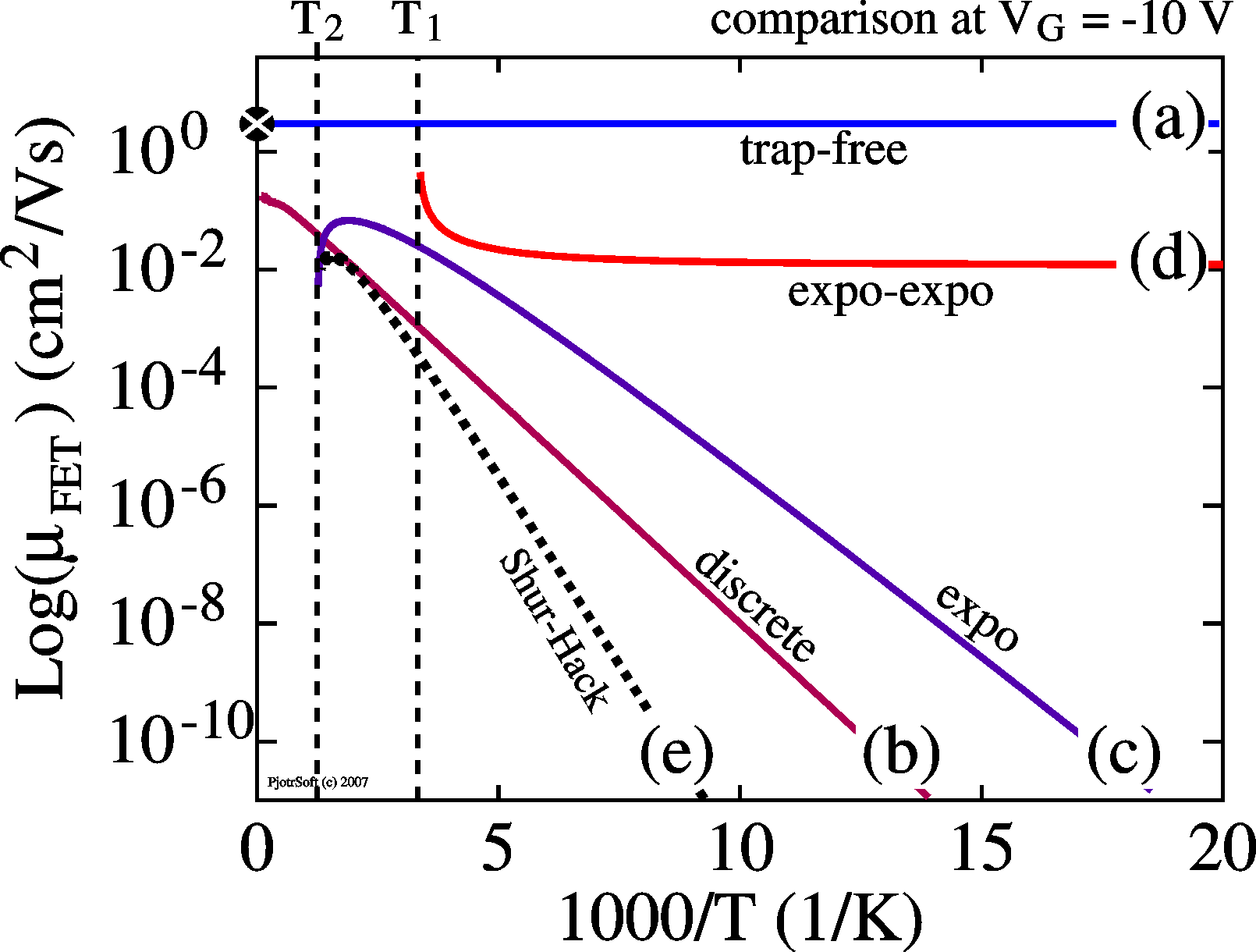Comparison of the various Arrhenius plots when governed by traps. Also the similar model of Shur and Hack for the 3-dimensional a-Si MOS-FET is shown. Organic Electronics 7, 592 (2006).

Effect of traps on current-transients
The traps manifest themselves in the current transients (Ids vs. t) in many time scales. Normally, the trapping speed is related to the trap depth,

t = exp(ET/kT)
• If the trapping is very fast, faster than the time it takes to take a single current sample, effectively, the device is always in thermal equilibrium and the mobility is temperature and bias dependent as shown above. The current is stable; Ids is independent of t. See Organic Electronics 7, 592 (2006).
• For intermediate timescales of trapping, care has to be taken in the measurements, since the I-V and transfer curves can show distortions (for instance hysteresis). This is very awkward since it is difficult to perform reliable and reproducible measurements. It is not possible to systematically study this. Especially organic materials suffer from this and data presented are often unreliable.
• If trapping is slow, the transients are exponential for a single discrete trap. For a huge density of traps, the final current is zero, since all charge is eventually trapped. For a distribution of traps, the time constants of the transients also become distributed according to the above equation. The resulting transient is then often of the stretched exponential type (J. Appl. Phys. 96, 5277 (2004)),
Ids ~ exp[ - (t/t)b ]

 Simple exponential Multi-exponential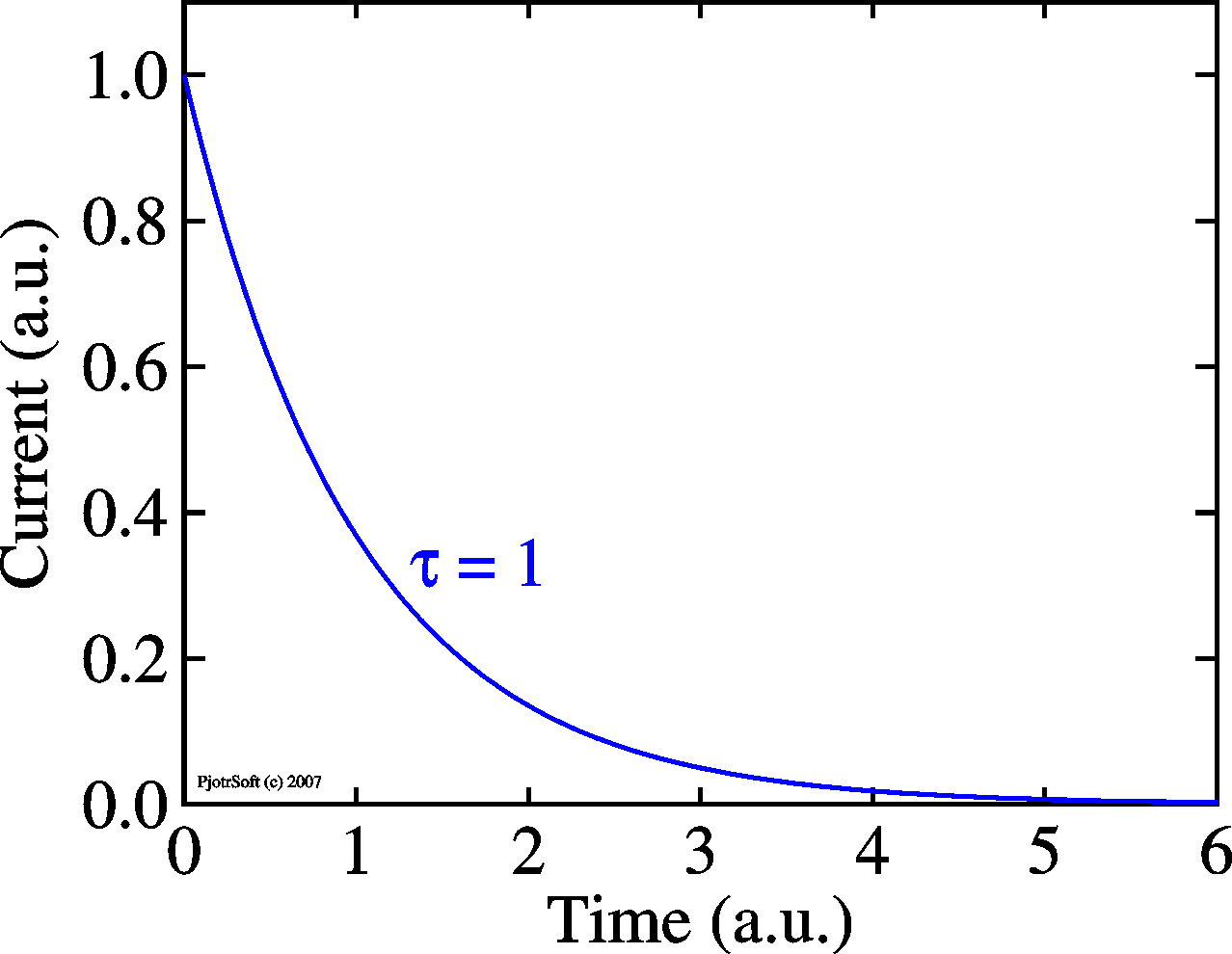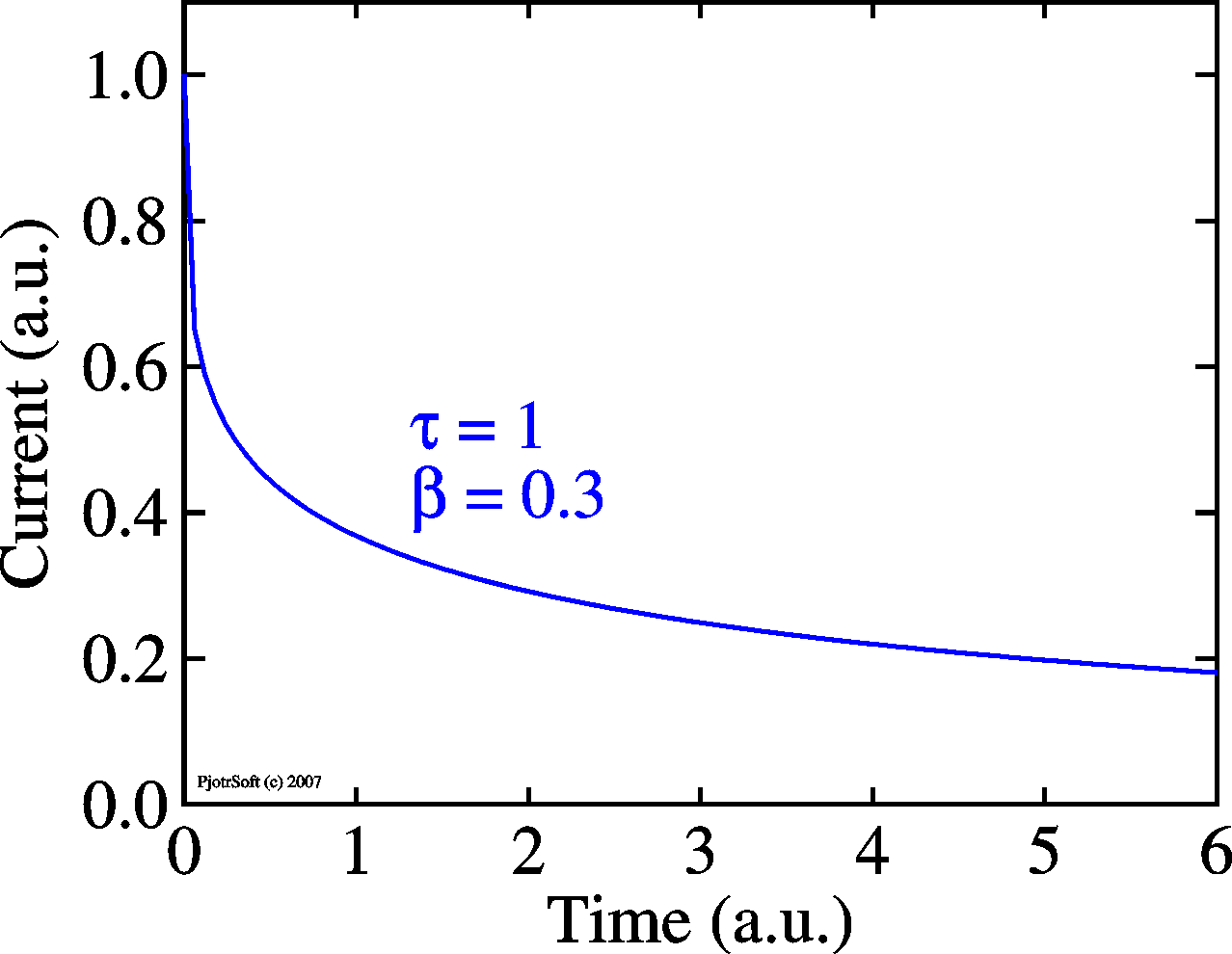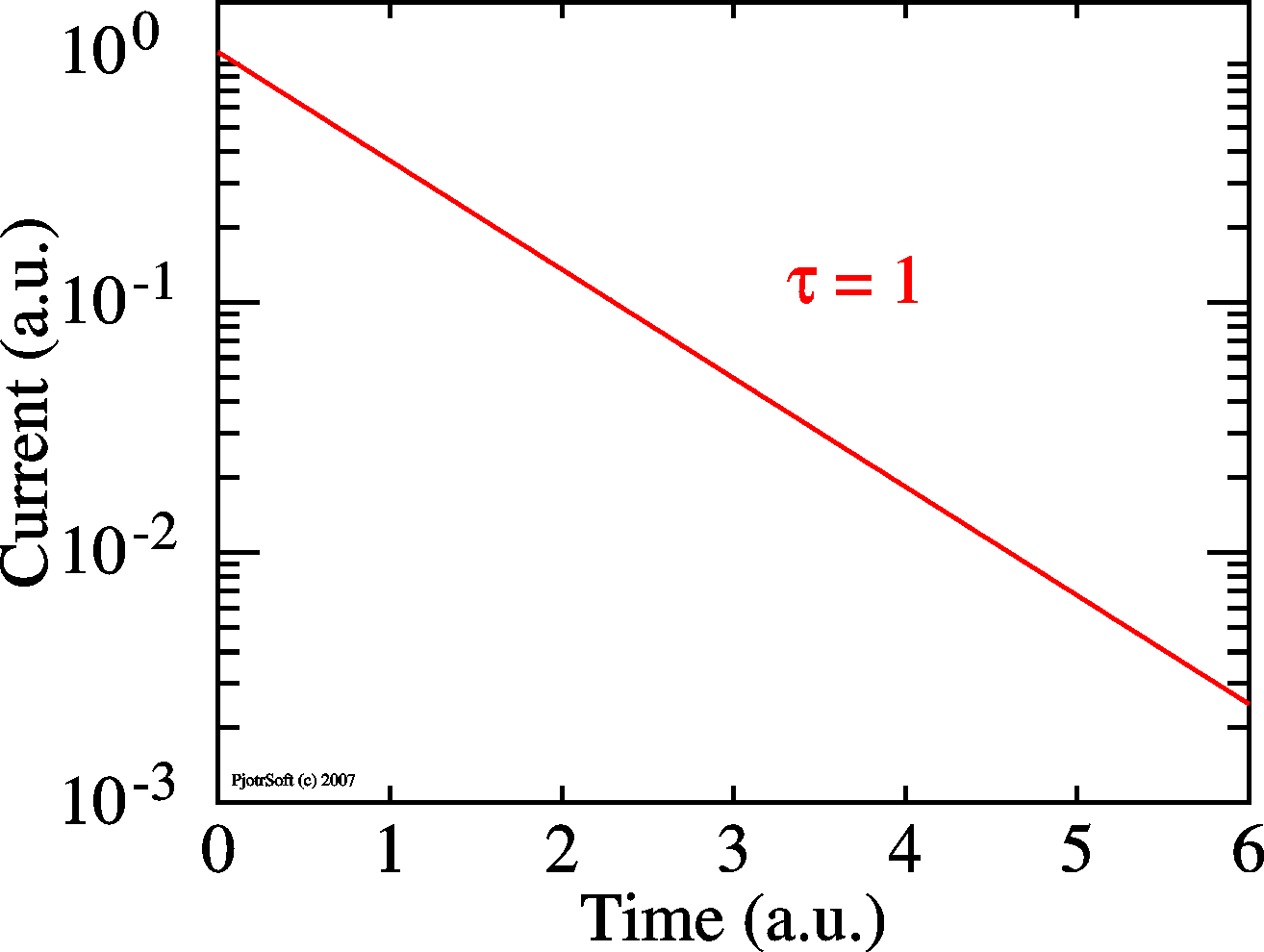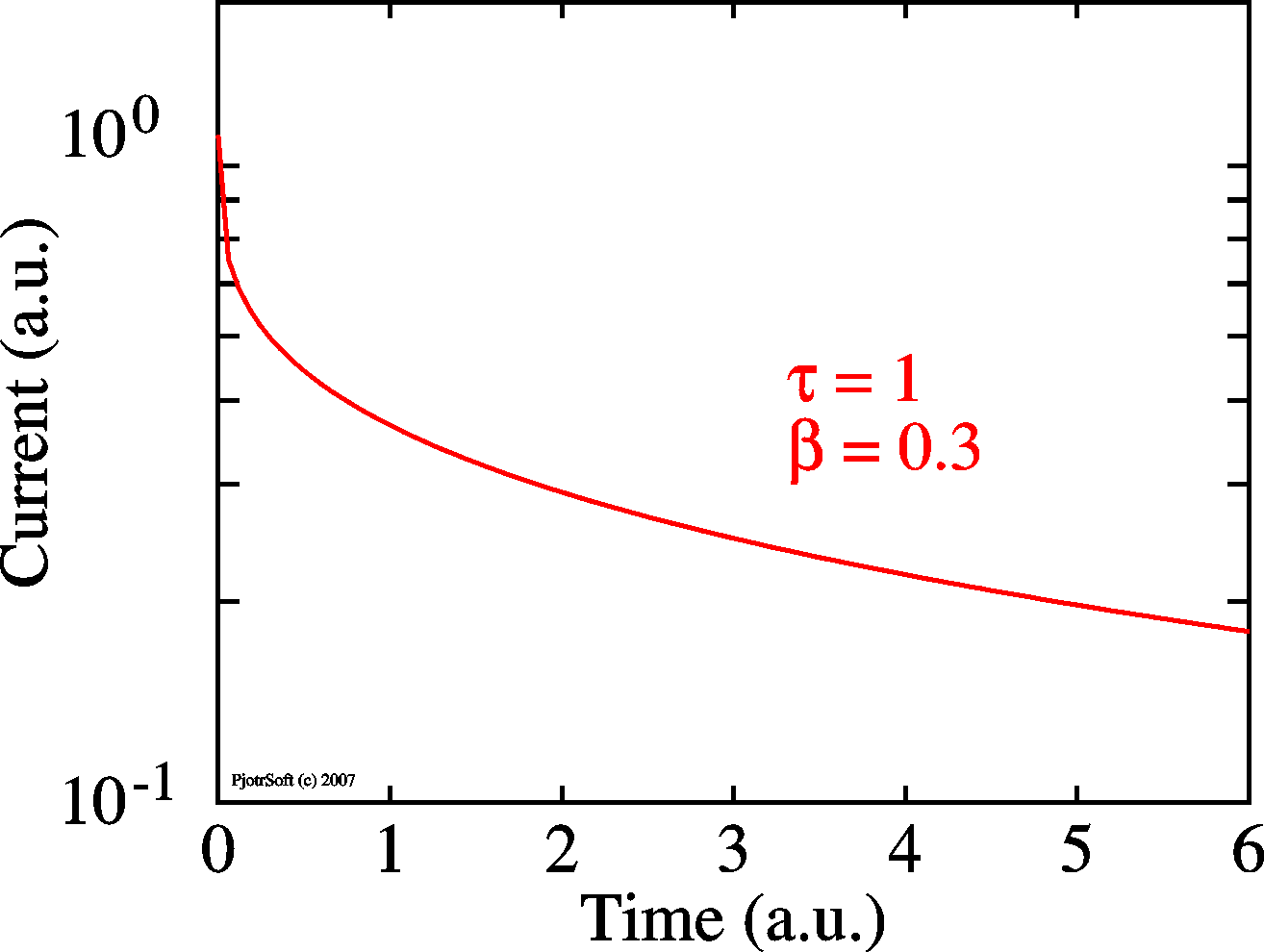• If trapping is extremely slow, the I-V and transfer curves are 'clean' during the measurements, but two consecutive measurements may look different. An effect called 'stressing' is the effect that Vt changes after prolonged application of bias, see Appl. Phys. Lett. 84, 3184 (2004). The threshold voltage Vth (= Vt) as a function of time is give as (Eth = kBT ln(nt).) Remarkably, this stressing only occurs for temperatures above about 200 K. The device seems to undergo a phase transistion there (J. Appl. Phys. 96, 5277 (2004)).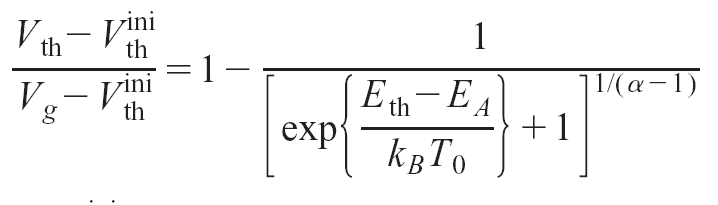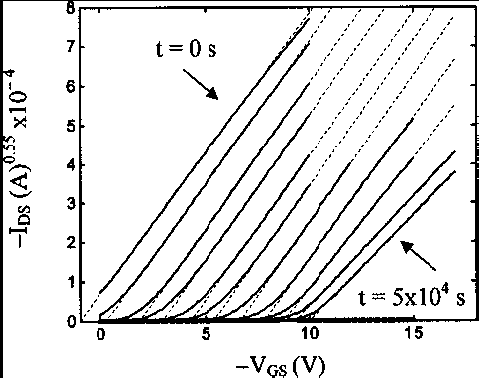The origin of the traps lies in the nature of the device. Depositing a thin film of material on a lattice-mismatching other material will unavoidably result in non-crystalline material, especially in layers close to the interface (exactly where the channel resides!). Recently we have discovered that water has a huge effect on the devices, see Appl. Phys. Lett. 88, 082101 (2006). The picture below shows the effect of exposure of an organic transistor to water. The picture shows the temperature scanned Ids current for small bias before and after exposure to water. In this way, the phase transition mentioned above could be ascribed to supercooled water.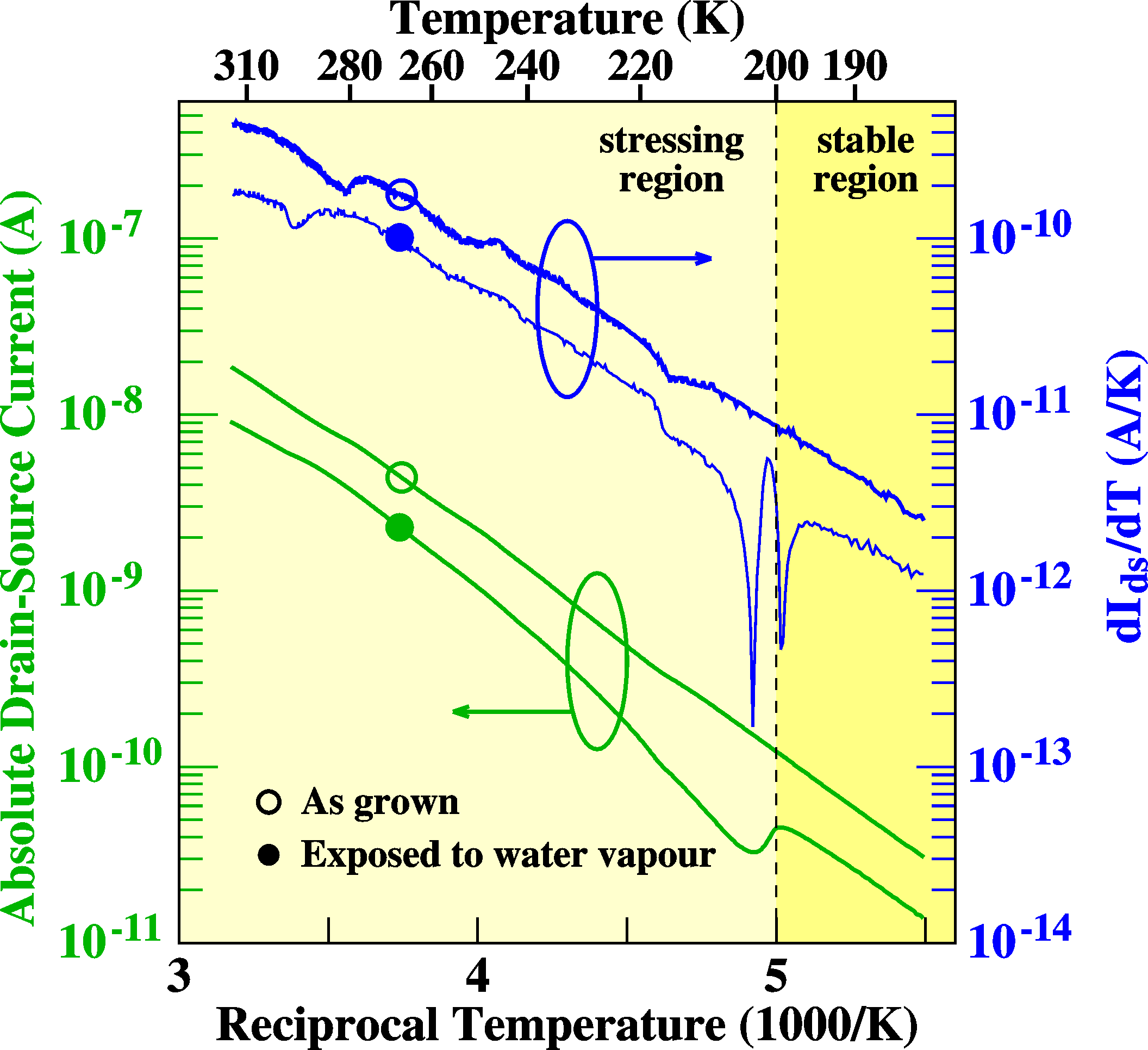## References

• Physics of semiconductor devices, S.M. Sze, 2nd edition, John Wiley & sons, 1981, ISBN 0-471-05661-8
• Physics of semiconductor devices, M. Shur, Prentice Hall, 1990, ISBN 0136664962
• Electrical Characterization of FETs of sexithiophene, P. Stallinga, H.L. Gomes, F. Biscarini, M. Murgia, J.Appl.Phys. (2004).
• Bias-induced threshold voltages shifts in thin-film organic transistors, H.L. Gomes, P. Stallinga, F. Dinelli, M. Murgia, F. Biscarini, D.M. de Leeuw, T. Muck, J. Geurts, L.W. Molenkamp, V. Wagner, Appl. Phys. Lett. 84, (2004).
• For the physical constants I relied on the very useful CERN pages
•        http://pdg.lbl.gov/2000/contents_sports.html,
It has the following official reference: C. Caso et al., The European Physical Journal C3 (1998) 1.page written and maintained by Peter Stallinga
Source written in HTML with Mozilla 1.7.5. Pictures made with PjotrSoft (my software name), output in PostScript format. Converted with Corel Draw  into GIFs and PNGs.
In no way were any electrons harmed in the process!